• 文章目录主成分分析主成分分析的基本原理总体主成分及其性质主成分主成分的性质相关问题讨论关于由协方差矩阵或相关矩阵出发求解主成分主成分分析不要求数据来自于正态总体主成分分析与重叠信息 主成分分析 主成分...
鄙人学习笔记

文章目录主成分分析主成分分析的基本原理总体主成分及其性质主成分主成分的性质相关问题讨论关于由协方差矩阵或相关矩阵出发求解主成分主成分分析不要求数据来自于正态总体主成分分析与重叠信息主成分分析步骤及逻辑框图步骤逻辑框图

主成分分析
主成分分析是利用降维的思想，在损失很少信息的前提下，把多个指标转化为几个综合指标的多元统计方法。
通常把转化生成的综合指标称为主成分，其中每个主成分都是原始变量的线性组合，且各个主成分之间互不相关，使得主成分比原始变量具有某些更优越的性能。
主成分分析的基本原理
在对某一事物进行实证研究时，为了更全面、准确地反映事物的特征及其发展规律，人们往往要考虑与其有关系的多个指标，这些指标在多元统计中也称为变量。这样就产生了如下问题：一方面人们为了避免遗漏重要的信息而考虑尽可能多的指标，另一方面考虑指标的增多增加了问题的复杂性，同时由于各指标均是对同一事物的反映，不可避免地造成信息的大量重叠，这种信息的重叠有时甚至会抹杀事物的真正特征与内在规律。基于上述问题，人们就希望在定量研究中涉及的变量较少，而得到的信息量又较多。
主成分分析正是研究如何通过原来变量的少数几个线性组合来解释原来变量绝大多数信息的一种多元统计方法。
既然研究某一问题涉及的众多变量之间有一定的相关性，就必然存在着起支配作用的共同因素。根据这一点，通过对原始变量相关矩阵或协方差矩阵内部结构关系的研究，利用原始变量的线性组合形成几个综合指标（主成分），在保留原始变量主要信息的前提下起到降维与简化问题的作用，使得在研究复杂问题时更容易抓住主要矛盾。

主成分分析的基本思想

利用主成分分析得到的主成分与原始变量之间有如下基本关系：
（1）每一个主成分都是各原始变量的线性组合。
（2）主成分的数目大大少于原始变量的数目。
（3）主成分保留了原始变量绝大多数信息。
（4）各主成分之间互不相关。

主成分分析的基本理论

对 X进行线性变换，可以形成新的综合变量，用 Y表示，也就是说，新的综合变量可以由原来的变量线性表示，即满足下式：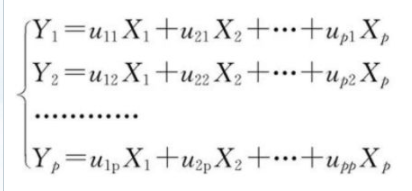由于可以任意地对原始变量进行上述线性变换，由不同的线性变换得到的综合变量Y 的统计特性也不尽相同。因此为了取得较好的效果，我们总是希望Yi=ui’X的方差尽可能大且各Yi 之间互相独立，由于: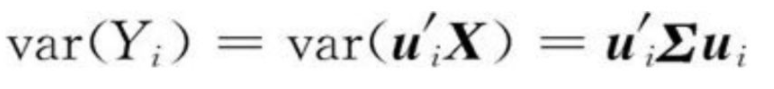对于任意的常数c,有：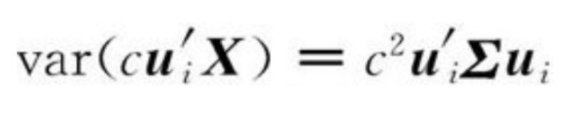因此对ui 不加限制时，可使var（Yi）任意增大，问题将变得没有意义。我们将线性变换约束在下面的原则之下：
（1）ui’ui=1（i ＝1，2，…，p）。
（2）Yi 与Yj 相互无关（i ≠ j；i，j ＝1，2，…，p）。
（3）Y1 是X1，X2，…，Xp 的一切满足原则(1)的线性组合中方差最大者；Y2 是与Y1 不相关的X1，X2，…，Xp所有线性组合中方差最大者；…，Yp 是与Y1，Y2，…，Yp－1 都不相关的X1，X2，…，Xp 的所有线性组合中方差最大者。
基于以上三条原则决定的综合变量 Y1， Y2，…， Yp分别称为原始变量的第一、第二……第 p个主成分。其中，各综合变量在总方差中所占的比重依次递减。

主成分分析的几何意义

主成分分析的过程无非就是坐标系旋转的过程，各主成分表达式就是新坐标系与原坐标系的转换关系，在新坐标系中，各坐标轴的方向就是原始数据变差最大的方向。
总体主成分及其性质
主成分分析的基本思想就是在保留原始变量尽可能多的信息的前提下达到降维的目的，从而简化问题的复杂性并抓住问题的主要矛盾。
而这里对于随机变量 X1， X2，…， Xp而言，其协方差矩阵或相关矩阵正是对各变量离散程度与变量之间的相关程度的信息的反映，而相关矩阵不过是将原始变量标准化后的协方差矩阵。
我们所说的保留原始变量尽可能多的信息，也就是指生成的较少的综合变量（主成分）的方差和尽可能接近原始变量方差的总和。因此在实际求解主成分的时候，总是从原始变量的协方差矩阵或相关矩阵的结构分析入手。
主成分

引论

设矩阵 A′＝ A，将 A的特征值 λ1， λ2，…， λn依大小顺序排列，不妨设 λ1 ≥ λ2 ≥… ≥ λn， γ1， γ2，…， γp为矩阵 A各特征值对应的标准正交特征向量，则对任意向量 x，有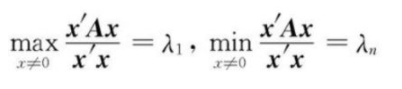从协方差阵出发求解主成分

设随机向量 X＝（ X1， X2，…， Xp）′的协方差矩阵为 ∑，  λ1 ≥ λ2 ≥… ≥ λn为 ∑的特征值，  γ1， γ2，…， γp为矩阵 A各特征值对应的标准正交特征向量，则第 i个主成分为：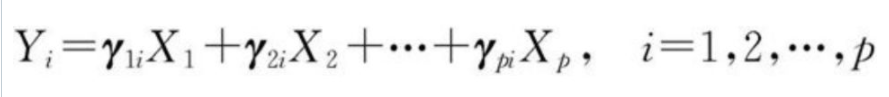此时：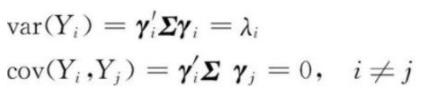Y的分量Y1，Y2，…，Yp依次是X的第一主成分、第二主成分……第p主成分的充分必要条件是：
（1）Y＝ P′ X，即 P为 p阶正交阵；
（2）Y的分量之间互不相关，即 D（ Y）＝ diag（ λ1， λ2，…， λp）；
（3）Y的 p个分量是按方差由大到小排列，即： λ1 ≥ λ2 ≥… ≥ λn
主成分的性质
性质1： Y的协方差阵为对角阵
性质2：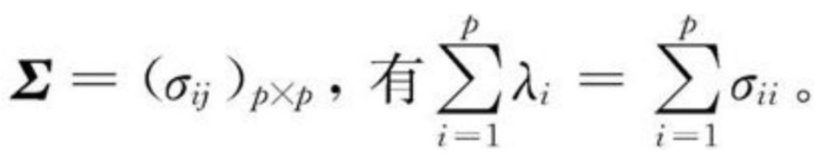累计贡献率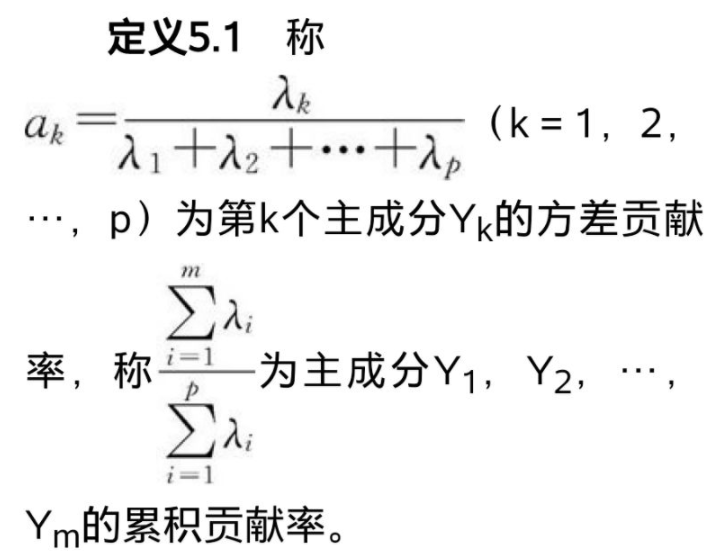主成分分析是把 p个随机变量的总方差分解为 p个不相关的随机变量的方差之和，使第一主成分的方差达到最大。
第一主成分是以变化最大的方向向量各分量为系数的原始变量的线性函数，最大方差为 λ1。
α1表明了 λ1的方差在全部方差中的比值，称 α1为第一主成分的贡献率。这个值越大，表明 Y1这个新变量综合 X1， X2，…， Xp信息的能力越强，也即由 Y1的差异来解释随机向量 X的差异的能力越强。
进行主成分分析的目的之一是减少变量的个数，所以一般不会取 p个主成分，而是取 m＜ p个主成分。 m取多少比较合适，是一个很实际的问题，通常以所取 m使得累积贡献率达到 85％以上为宜。

因子载荷量

第 k个主成分 Yk与原始变量 Xi的相关系数 ρ（ Yk， Xi）称为因子负荷量。
因子负荷量的绝对值大小刻画了该主成分的主要意义及其成因。
性质3：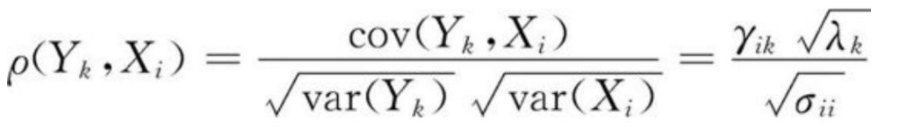因子负荷量 ρ（Yk， Xi）与系数 γik成正比，与 Xi的标准差成反比关系，因此，绝不能将因子负荷量与系数向量混为一谈。在解释主成分的成因或第 i个变量对第 k个主成分的重要性时，应当根据因子负荷量而不能仅仅根据 Yk与 Xi的变换系数 γik。
性质4：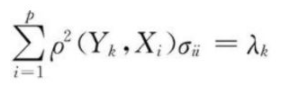性质5：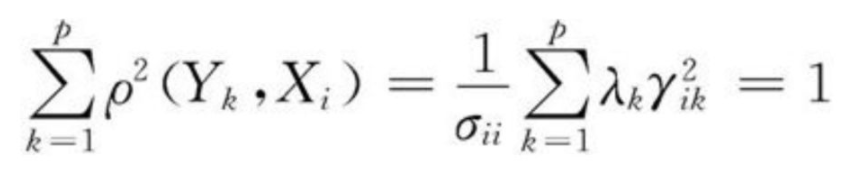Xi与 Y1， Y2，…， Yp的全相关系数的平方和等于 1。

方差贡献率vi

Xi与前 m个主成分 Y1， Y2，…， Ym的全相关系数平方和称为 Y1， Y2，…， Ym对原始变量 Xi的方差贡献率 vi，即：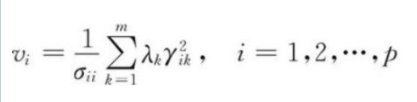这一定义说明了前 m个主成分提取了原始变量 Xi中 vi的信息，由此可以判断我们提取的主成分说明原始变量的能力。

从相关矩阵出发求解主成分

考虑如下的数学变换：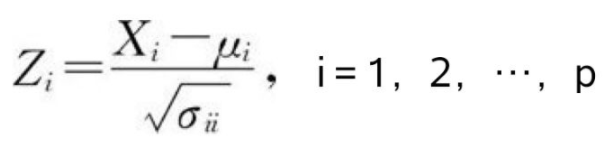式中，μi与σii分别表示变量Xi的期望与方差。
于是有 E（Zi）＝0，　　var（Zi）＝1
原始变量 X1， X2，…， Xp的相关阵实际上就是对原始变量标准化后的协方差矩阵。因此，由相关矩阵求主成分的过程与主成分个数的确定准则实际上是与由协方差矩阵出发求主成分的过程与主成分个数的确定准则相一致的。

由相关性求主成分时主成分的简单形式

（1）Y的协方差矩阵为对角阵ʌ。
（2）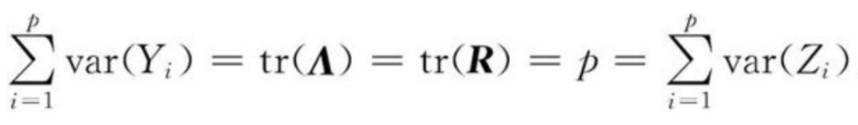（3）第 k个主成分的方差占总方差的比例，即第 k个主成分的方差贡献率为 αk＝ λk/ p，前 m个主成分的累积方差贡献率为：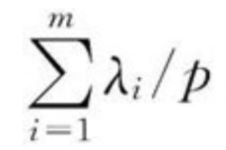（4）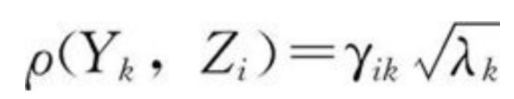相关问题讨论
关于由协方差矩阵或相关矩阵出发求解主成分
求解主成分的过程实际就是对矩阵结构进行分析的过程，也就是求解特征值的过程。从协方差阵出发和从相关阵出发所求得的主成分一般来说是有差别的，而且这种差别有时候还很大。
一般而言，对于度量单位不同的指标或取值范围彼此差异非常大的指标，不直接由其协方差矩阵出发进行主成分分析，而应该考虑将数据标准化。
但是，对原始数据进行标准化处理后倾向于各个指标的作用在主成分的构成中相等。对于取值范围相差不大或度量相同的指标进行标准化处理后，其主成分分析的结果仍与由协方差阵出发求得的结果有较大区别。
其原因是由于对数据进行标准化的过程实际上也就是抹杀原始变量离散程度差异的过程，标准化后的各变量方差相等均为 1，而实际上方差也是对数据信息的重要概括形式，也就是说，对原始数据进行标准化后抹杀了一部分重要信息，因此才使得标准化后各变量在对主成分构成中的作用趋于相等。
由此看来，对同度量或取值范围在同量级的数据，还是直接从协方差矩阵求解主成分为宜。
主成分分析不要求数据来自于正态总体
也就是说，与很多多元统计方法不同，主成分分析不要求数据来自于正态总体。实际上，主成分分析就是对矩阵结构的分析，其中主要用到的技术是矩阵运算的技术及矩阵对角化和矩阵的谱分解技术。我们知道，对多元随机变量而言，其协方差矩阵或相关矩阵均是非负定的，这样，就可以按照求解主成分的步骤求出其特征值、标准正交特征向量，进而求出主成分，达到缩减数据维数的目的。
主成分分析与重叠信息
首先应当认识到，主成分分析方法适用于变量之间存在较强相关性的数据，如果原始数据相关性较弱，运用主成分分析后不能起到很好的降维作用，即所得的各个主成分浓缩原始变量信息的能力差别不大。
一般认为，当原始数据大部分变量的相关系数都小于 0. 3时，运用主成分分析不会取得很好的效果。
很多研究工作者在运用主成分分析方法时，都或多或少存在着对主成分分析消除原始变量重叠信息的期望，这样，在实际工作之初就可以把与某一研究问题相关而可能得到的变量（指标）都纳入分析过程，再用少数几个主成分浓缩这些有用信息（假定已剔除了重叠信息），然后对主成分进行深入分析。
在对待重叠信息方面，生成的新的综合变量（主成分）是有效剔除了原始变量中的重叠信息，还是仅按原来的模式将原始信息中的绝大部分用几个不相关的新变量表示出来，这一点还有待讨论。

多重共线性

在实际工作中，在选取初始变量进入分析时应该小心，对原始变量存在多重共线性的问题，在应用主成分分析方法时一定要慎重。应该考虑所选取的初始变量是否合适，是否真实地反映了事物的本来面目，如果是出于避免遗漏某些信息而特意选取了过多的存在重叠信息的变量，就要特别注意应用主成分分析所得到的结果。
如果所得到的样本协方差矩阵(或者相关阵)最小特征值接近于0，那么就意味着，中心化以后的原始变量之间存在着多重共线性，即原始变量存在着不可忽视的重叠信息。
因此，在进行主成分分析得出协方差阵或是相关阵，发现最小特征根接近于零时，应该注意对主成分的解释，或者考虑对最初纳入分析的指标进行筛选。由此可以看出，虽然主成分分析不能有效地剔除重叠信息，但它至少可以发现原始变量是否存在重叠信息，这对减少分析中的失误是有帮助的。
主成分分析步骤及逻辑框图
步骤
进行主成分分析的步骤，对此进行归纳如下：
（1）根据研究问题选取初始分析变量；
（2）根据初始变量特性判断由协方差阵求主成分还是由相关阵求主成分；
（3）求协方差阵或相关阵的特征根与相应标准特征向量；
（4）判断是否存在明显的多重共线性，若存在，则回到第一步；
（5）得到主成分的表达式并确定主成分个数，选取主成分；
（6）结合主成分对研究问题进行分析并深入研究。
逻辑框图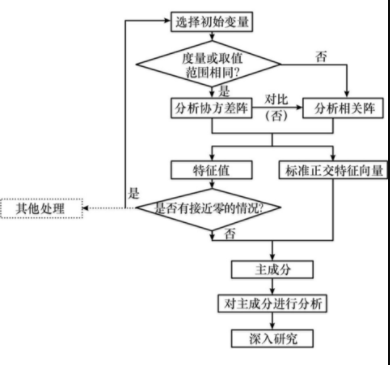展开全文多元统计
• 目录1 总体主成分1.1 主成分的定义与导出1.2 主成分的性质1.3 从相关矩阵出发求主成分2 样本主成分2.1 从S出发求主成分2.2 从R出发求主成分3 相关的R函数以及实例3.1 princomp函数3.2 summary函数3.3 loadings...
目录1 总体主成分1.1 主成分的定义与导出1.2 主成分的性质1.3 从相关矩阵出发求主成分2 样本主成分2.1 从S出发求主成分2.2 从R出发求主成分3 相关的R函数以及实例3.1 princomp函数3.2 summary函数3.3 loadings函数3.4 predict函数3.5 screeplot函数3.6 biplot函数4 实例附录——PCA高级散点图的绘制补充说明
主成分分析（principal component analysis）是将多指标化为少数几个综合指标的一种统计分析方法，是由Pearson（1901）提出，后来被Hotelling（1933）发展了。主成分分析是一种通过降维技术把多个变量化成少数几个主成分的方法.这些主成分能够发映原始变量的绝大部分信息，它们通常表示为原始变量的线性组合.
1 总体主成分
1.1 主成分的定义与导出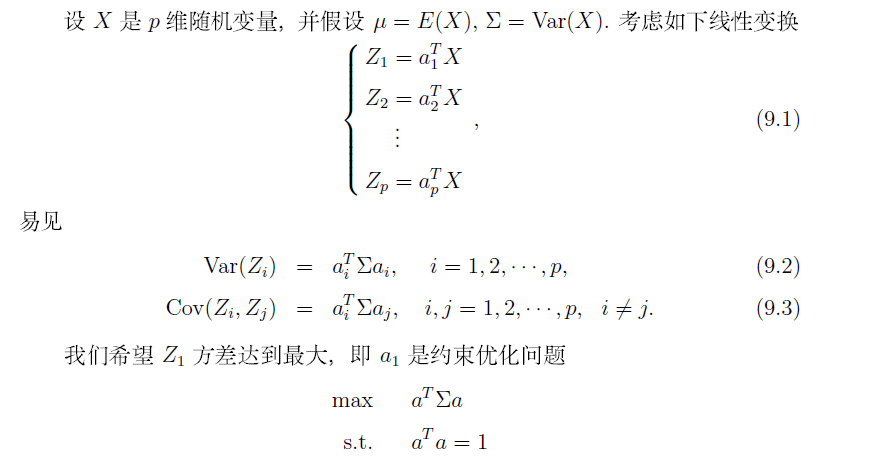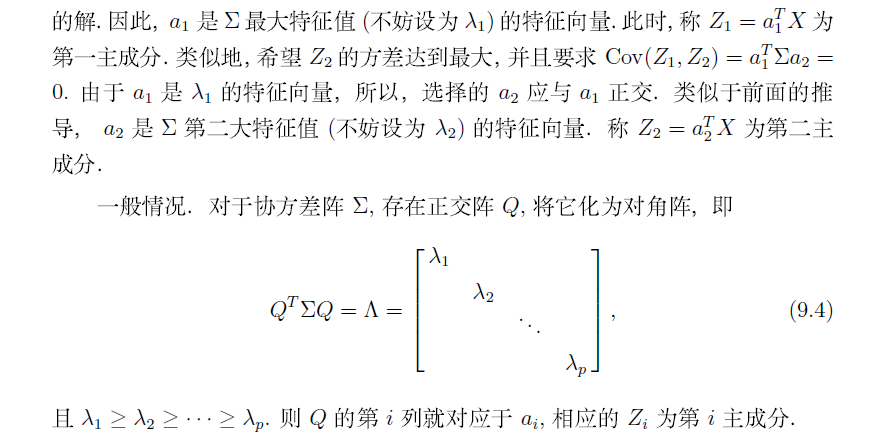1.2 主成分的性质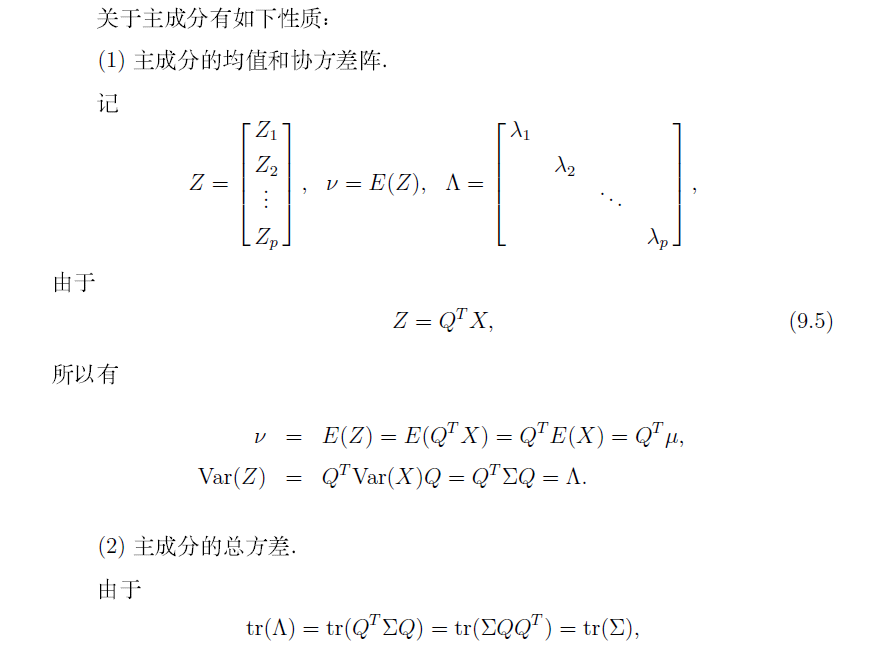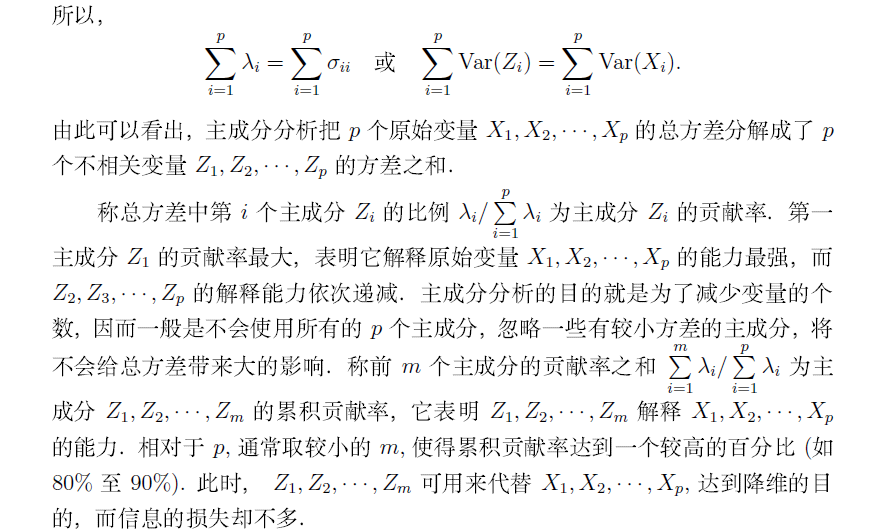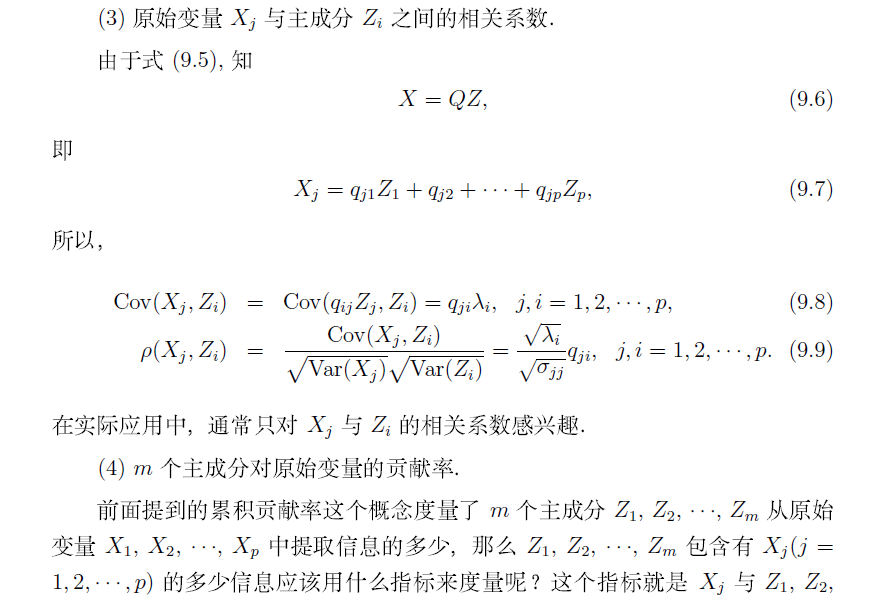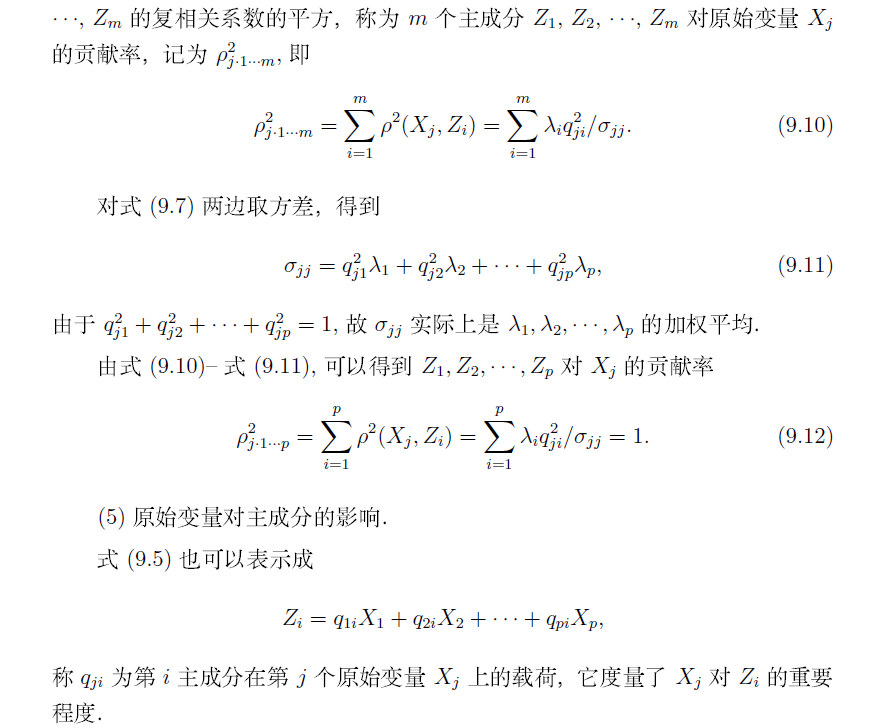1.3 从相关矩阵出发求主成分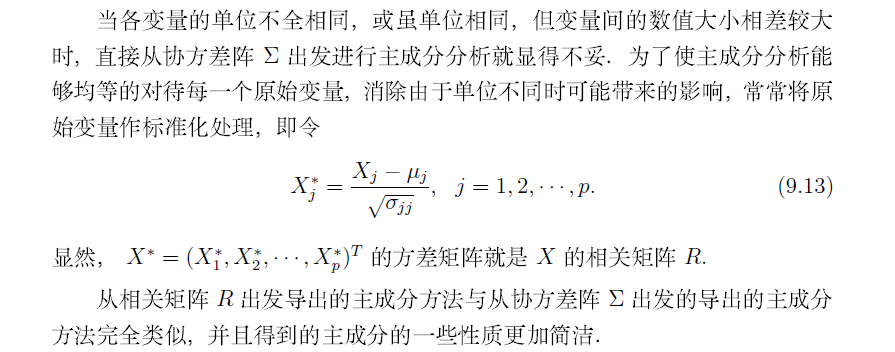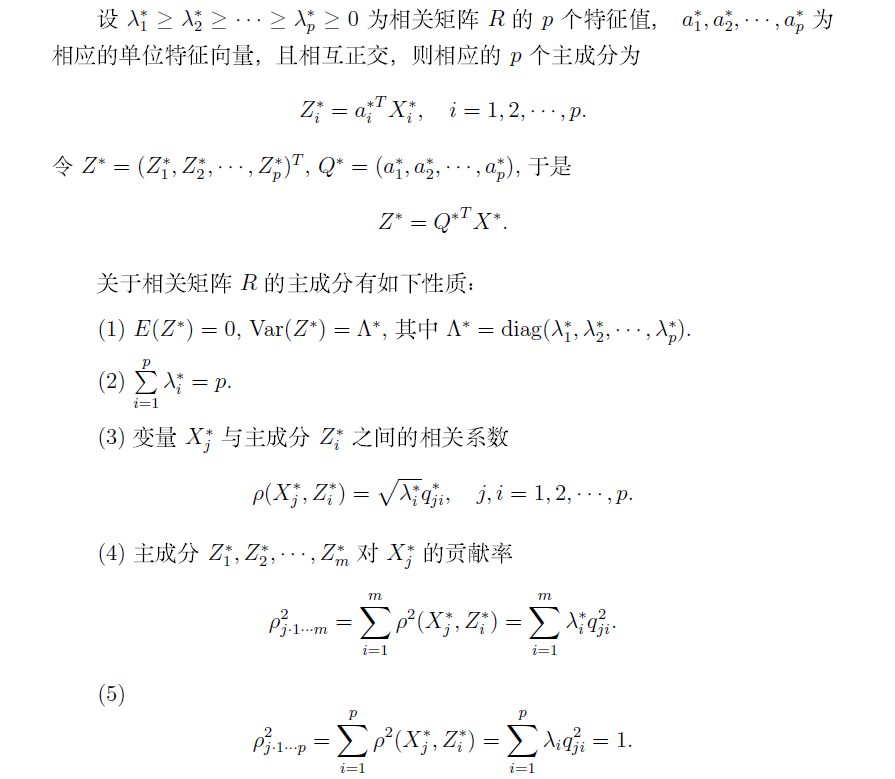2 样本主成分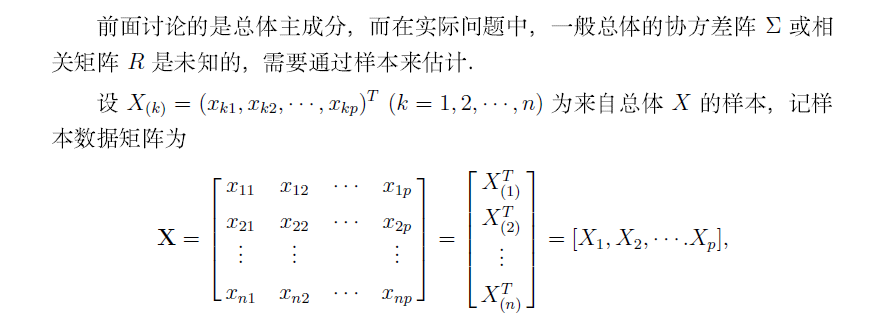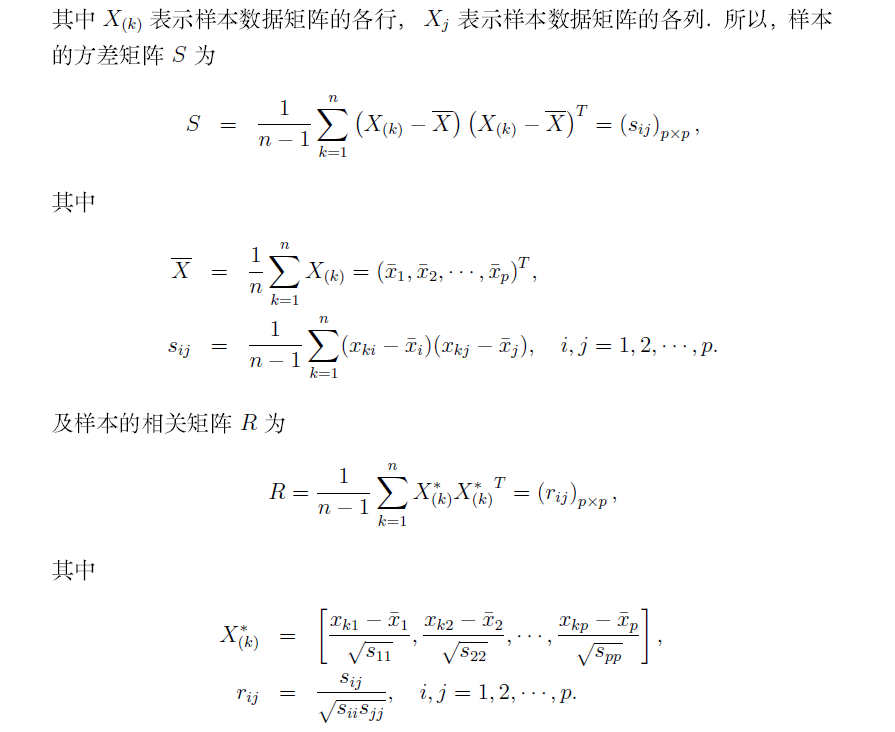2.1 从S出发求主成分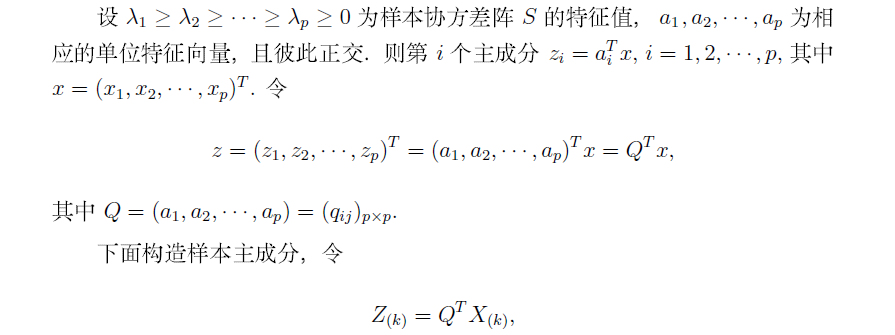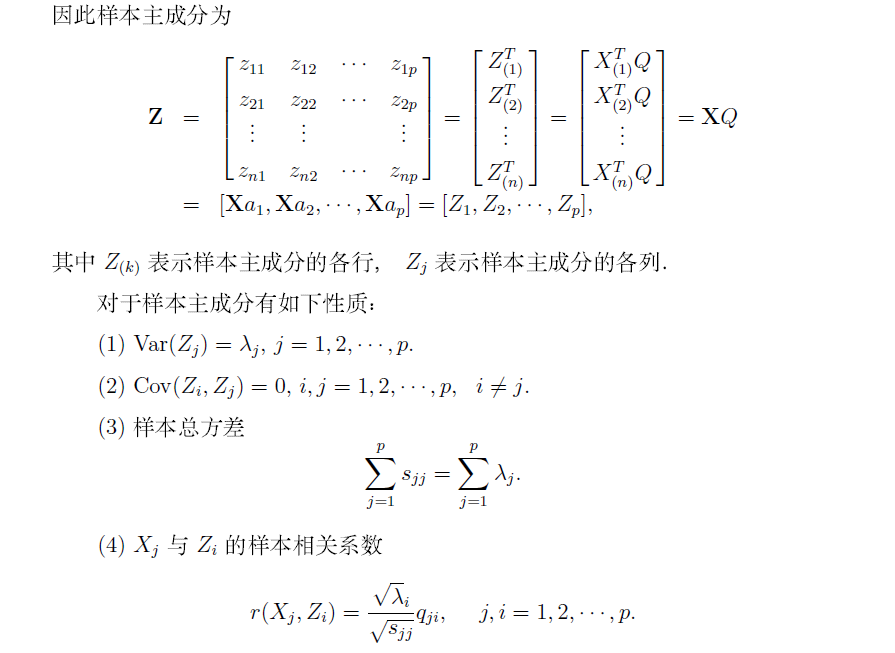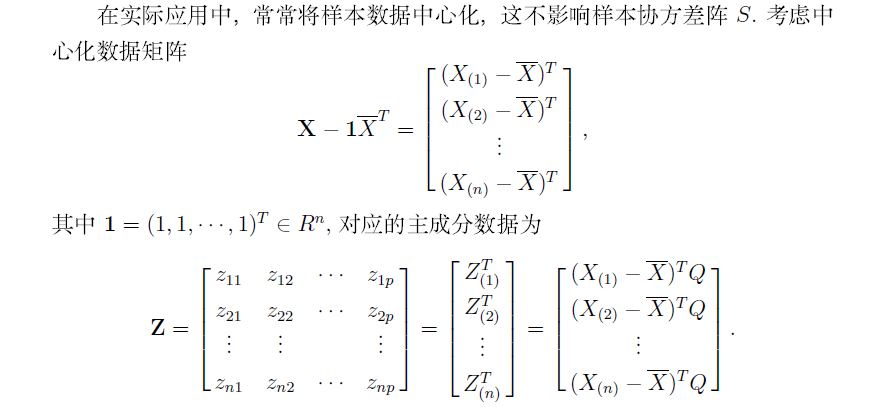2.2 从R出发求主成分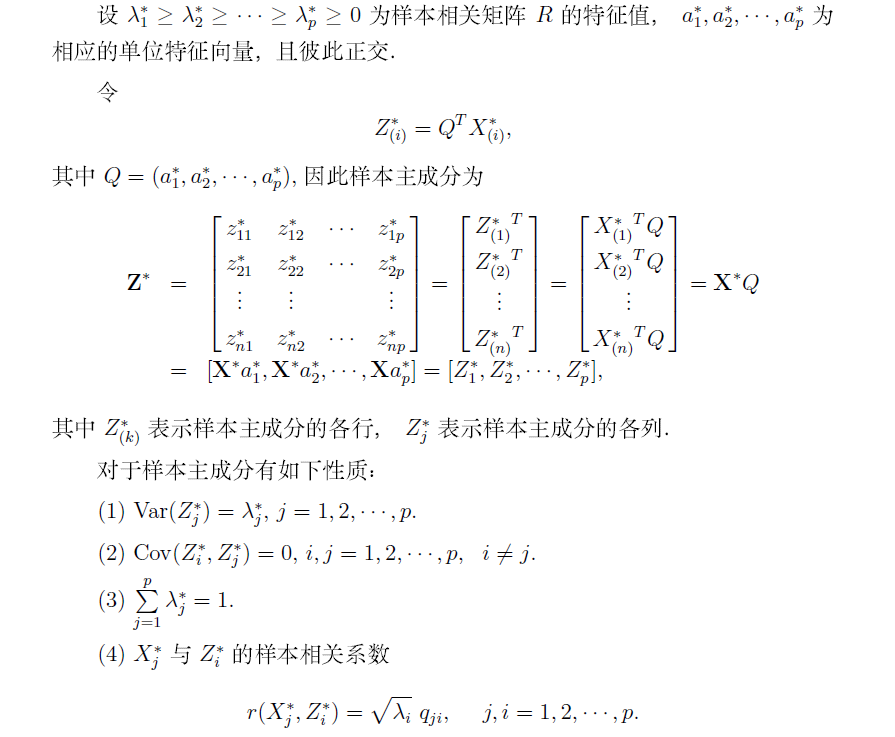3 相关的R函数以及实例
下面介绍与主成分分析有关的函数：
3.1 princomp函数
作主成分分析最主要的函数是princomp()函数，其使用格式为:

princomp(formula, data = NULL, subset, na.action, ...)

其中formula是没有响应变量的公式（类似回归分析、方差分析，但无响应变量）。data是数据框（类似于回归分析、方差分析）。或者

princomp(x, cor = FALSE, scores = TRUE, covmat = NULL, subset = rep(TRUE, nrow(as.matrix(x))), ...)

其中x是用于主成分分析的数据，以数值矩阵或数据框的形式给出.cor是逻辑变量，当cor=TRUE表示用样本的相关矩阵R作主成分分析，当cor=FALSE（缺省值）表示用样本的协方差阵S作主成分分析。covmat是协方差阵，如果数据不用x提供，可由协方差阵提供.其他参数的意义见在线帮助.
prcomp()函数的意义与使用方法与princomp()函数相同.
3.2 summary函数
summary()与回归分析中的用法相同，其目的是提取主成分的信息，其作用格式为

summary(object, loadings = FALSE, cutoff = 0.1, ...)

其中object是由princomp()得到的对象。loadings是逻辑变量，当loadings=TRUE表示显示loadings的内容（具体含义在下面的loadings()函数），当loadings=FALSE则不显示.
3.3 loadings函数
loadings()函数是显示主成分分析或因子分析中loadings（载荷）的内容。在主成分分析中，该内容实际上是主成分对应的各列，即前面分析的正交矩阵Q。在因子分析中，其内容就是载荷因子矩阵。loadings()函数的使用格式为

loadings(x)

其中x是由函数princomp()或factanal()（见因子分析）得到的对象。
3.4 predict函数
predict()函数是预测主成分的值（类似于回归分析中的使用方法），其使用格式为

predict(object, newdata, ...)

其中object是由princomp()得到的对象.newdata是由预测值构成的数据框，当newdata缺省时，预测已有数据的主成分值.
3.5 screeplot函数
screeplot()函数是画出主成分的碎石图，其使用格式为

screeplot(x, npcs = min(10, length(x$sdev)), type=c("barplot", "lines"), main = deparse(substitute(x)), ...) 其中x是由princomp()得到的对象。npcs是画出的主成分的个数。type是描述画出的碎石图的类型，"barplot"是直方图类型，“lines"是直线图类型. 3.6 biplot函数 biplot()是画出数据关于主成分的散点图和原坐标在主成分下的方向，其使用格式为 biplot(x, choices=1:2, scale=1, pc.biplot=FALSE,...) 其中x是由princomp()得到的对象。choices是选择的主成分，缺省值是第1、第2主成分。pc.biplot是逻辑变量（缺省值为FALSE），当pc.biplot=TRUE，用Gabriel（1971）提出的画图方法.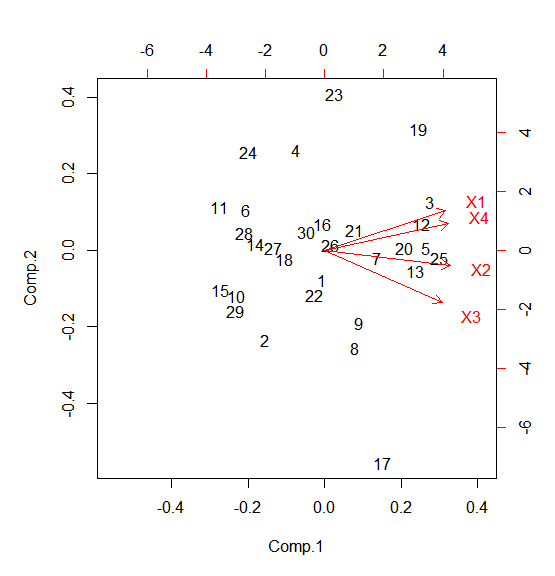4 实例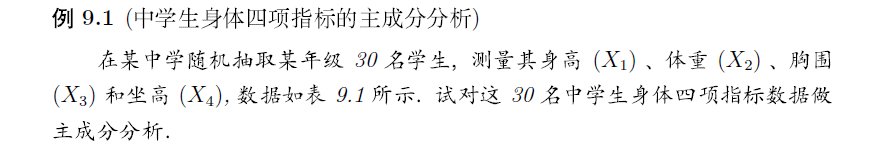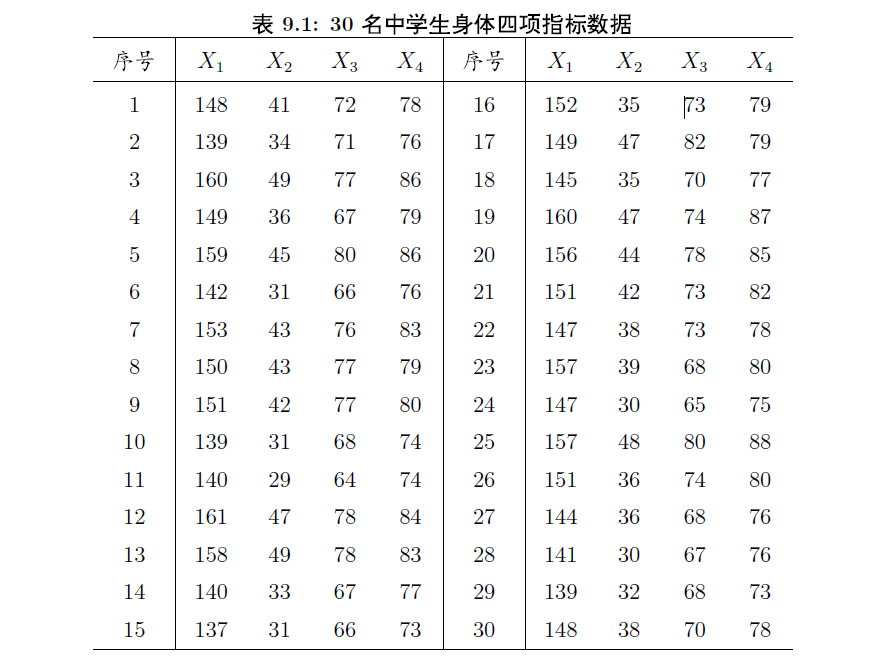## 用相关矩阵做主成分分析，并显示分析结果 student.pr <- princomp(student, cor=TRUE) summary(student.pr, loadings=TRUE) # 显示载荷矩阵 Importance of components: Comp.1 Comp.2 Comp.3 Comp.4 Standard deviation 1.8817805 0.55980636 0.28179594 0.25711844 # R的特征值的开放 Proportion of Variance 0.8852745 0.07834579 0.01985224 0.01652747 # 方差贡献率 Cumulative Proportion 0.8852745 0.96362029 0.98347253 1.00000000 # 累计贡献率 Loadings: # R的特征向量 Comp.1 Comp.2 Comp.3 Comp.4 X1 0.497 0.543 0.450 0.506 X2 0.515 -0.210 0.462 -0.691 X3 0.481 -0.725 -0.175 0.461 X4 0.507 0.368 -0.744 -0.232  在上述程序中，语句student.pr<-princomp(student，cor=TRUE)可以改成student.pr<-princomp(~X1+x2+X3+X4，data=student，cor=TRUE)，两者是等价的. summary()函数列出了主成分分析的重要信息， Standard deviation行表示的是主成分的标准差，即主成分的方差的开方，也就是相关阵的特征值$\lambda_1$,$\lambda_2$,$\lambda_3$,$\lambda_4$的开方. Proportion of Variance行表示的是方差的贡献率. Cumulative Proportion行表示的是方差的累积贡献率. 第1主成分对应系数的符号都相同，其值在0.5左右，它反映了中学生身材魁梧程度：身体高大的学生，他的4个部分的尺寸都比较大，因此，第1主成分的值就较小（因为系数均为负值）；而身材矮小的学生，他的4部分的尺寸都比较小，因此，第1主成分绝对值就较大.我们称第1主成分为大小因子.第2主成分是高度与围度的差，第2主成分值大的学生表明该学生“细高”，而第2主成分值越小的学生表明该学生“矮胖”，因此，称第2主成分为体形因子. 我们看一下各样本的主成分的值（用predict()函数）. ## 查看主成分 predict(student.pr) Comp.1 Comp.2 Comp.3 Comp.4 [1,] -0.06990950 -0.23813701 0.35509248 -0.266120139 [2,] -1.59526340 -0.71847399 -0.32813232 -0.118056646 [3,] 2.84793151 0.38956679 0.09731731 -0.279482487 [4,] -0.75996988 0.80604335 0.04945722 -0.162949298 [5,] 2.73966777 0.01718087 -0.36012615 0.358653044 [6,] -2.10583168 0.32284393 -0.18600422 -0.036456084 [7,] 1.42105591 -0.06053165 -0.21093321 -0.044223092 [8,] 0.82583977 -0.78102576 0.27557798 0.057288572 [9,] 0.93464402 -0.58469242 0.08814136 0.181037746 [10,] -2.36463820 -0.36532199 -0.08840476 0.045520127 [11,] -2.83741916 0.34875841 -0.03310423 -0.031146930 [12,] 2.60851224 0.21278728 0.33398037 0.210157574 [13,] 2.44253342 -0.16769496 0.46918095 -0.162987830 [14,] -1.86630669 0.05021384 -0.37720280 -0.358821916 [15,] -2.81347421 -0.31790107 0.03291329 -0.222035112 [16,] -0.06392983 0.20718448 -0.04334340 0.703533624 [17,] 1.55561022 -1.70439674 0.33126406 0.007551879 [18,] -1.07392251 -0.06763418 -0.02283648 0.048606680 [19,] 2.52174212 0.97274301 -0.12164633 -0.390667991 [20,] 2.14072377 0.02217881 -0.37410972 0.129548960 [21,] 0.79624422 0.16307887 -0.12781270 -0.294140762 [22,] -0.28708321 -0.35744666 0.03962116 0.080991989 [23,] 0.25151075 1.25555188 0.55617325 0.109068939 [24,] -2.05706032 0.78894494 0.26552109 0.388088643 [25,] 3.08596855 -0.05775318 -0.62110421 -0.218939612 [26,] 0.16367555 0.04317932 -0.24481850 0.560248997 [27,] -1.37265053 0.02220972 0.23378320 -0.257399715 [28,] -2.16097778 0.13733233 -0.35589739 0.093123683 [29,] -2.40434827 -0.48613137 0.16154441 -0.007914021 [30,] -0.50287468 0.14734317 0.20590831 -0.122078819  从第1主成分来看，较小的几个值是25号样本、3号样本和5号样本，因此说明这几个学生身材魁梧；而11号样本、15号样本和29号样本的的值较大，说明这几个学生身材瘦小. 从第2主成分来看，较大的几个值是23号样本、19号样本和4号样本，因此说明这几个学生属于“细高”型；而17号样本、8号样本和2号样本的的值较小，说明这几个学生身材属于“矮胖”型. 画出主成分的碎石图，参数选择直线型，可以换为直方图： ## 主成分的碎石图 screeplot(student.pr, type=c("lines"))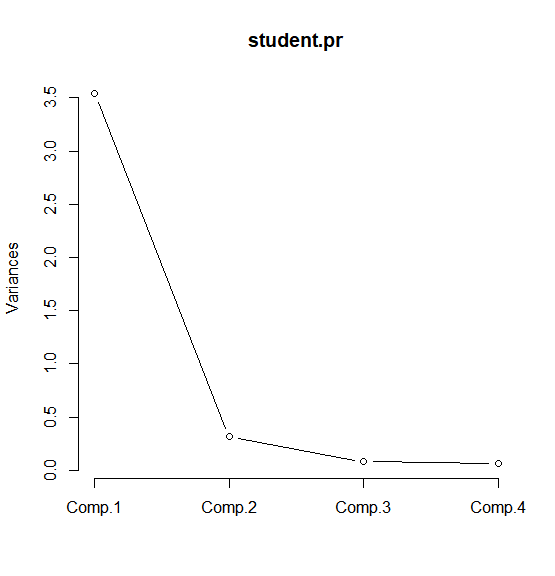还可以画出关于第1主成分和第2主成分样本的散点图，其图形如下图所示.从该散点图可以很容易看出：哪些学生属于高大魁梧型，如25号学生，哪些学生属于身材瘦小型，如11号或15号；哪些学生属于“细高”型，如23号，哪些学生属于“矮胖”型，如17号.还有哪些学生属于正常体形，如26号，等等. 附录——PCA高级散点图的绘制 使用ggplot2绘制PCA散点图 分别利用颜色（colour）和形状（shape i.e. pch）进行分组很多属性需要单独设置。 用到的对象有 数据映射（Aes，Data aesthetic mappings） 几何属性（Geom，Geometric objects） 统计转换（Stat，Statistical transformations） 标度（Scales） 坐标系（xlab, ylab, coor, coordinate system） 主题（theme） 暂时未用到分面（faceting） myData <- read.csv("data.csv") myData2 <- myData[,-20] myData3 <- na.omit(myData2) pr<-prcomp(myData3[,5:20],scale=TRUE) pca <- pr$x

####一下为ggplot2绘图

library(ggplot2)
pca <- as.data.frame(pca)
pca$salinity <- myData3$Salinity
pca$climate <- myData3$Climate
pca$ecotype <- myData3$Ecotype
pca$name <- myData3$Plant.name

theme<-theme(panel.background = element_blank(),
panel.border=element_rect(fill=NA),
panel.grid.major = element_blank(),
panel.grid.minor = element_blank(),
strip.background=element_blank(),
axis.text.x=element_text(colour="black"),
axis.text.y=element_text(colour="black"),
axis.ticks=element_line(colour="black"),
plot.margin=unit(c(1,1,1,1),"line"),
legend.title=element_blank(),
legend.key = element_rect(fill = "white")
)
percentage <- round(pr$sdev / sum(pr$sdev) * 100, 2)
percentage <- paste( colnames(pca), "(", paste( as.character(percentage), "%", ")", sep="") )

p <- ggplot(pca, aes(x=PC1, y=PC2,shape=paste(salinity, climate), colour=ecotype,label=name))
#p <- p + geom_point()+geom_text(size=3) + theme

p <- p + geom_point(size=3) +
theme +
xlab(percentage)  +
ylab(percentage)  +
scale_shape_manual(values = c(15,17,0,2),labels=c("Salt in Fanggan","Salt in Panjin","Fresh in Fanggan","Fresh in Panjin"))+
stat_ellipse(aes(shape = NULL))+
scale_x_continuous(limits = c(-6,6))+
scale_y_continuous(limits = c(-5,5.5))
p

结果：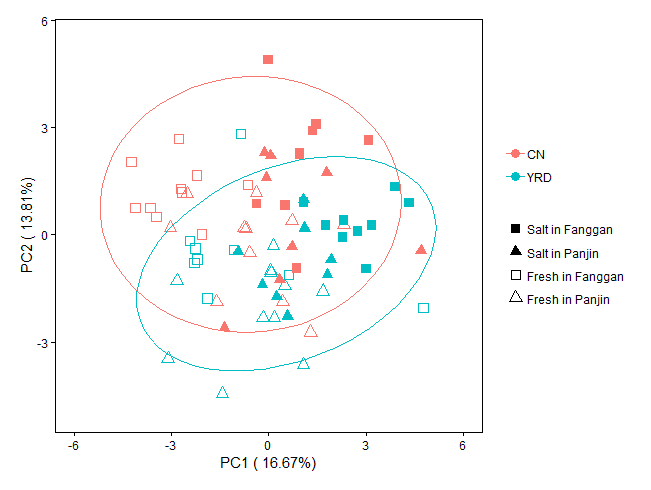补充说明
主成分分析是只能针对连续变量来进行压缩，分类变量则不行。 因为分类变量之间可以说是完全独立的，并没有正负两种相关性一说，如性别男和女之间就完全是独立的。如果一定也要将分类变量压缩的话，通常会对他们进行WOE转换，之后就可以愉快的进行压缩了。所以分类变量是没办法进行单独压缩的，因为没有对应的算法。有些人可能会直接对分类变量间进行卡方检验，然后把 p 值大的删去一些，这个其实应该被划分为手工的范畴，并不属于算法。


展开全文机器学习 数据分析 数据挖掘
• 第十六章 主成分分析（PCA）摘要1 总体主成分分析1.1 基本思想1.2 总体主成分分析定义1.3 主成分的个数1.4 规范化变量的总体主成分分析2 样本主成分分析2.1 样本主成分的定义和性质 摘要 主成分分析（Principal ...
第十六章 主成分分析（PCA）摘要1 总体主成分分析1.1 基本思想1.2 总体主成分分析定义1.3 主成分的个数1.4 规范化变量的总体主成分分析2 样本主成分分析2.1 样本主成分的定义和性质2.2 主成分分析方法2.2.1 相关矩阵的特征值分解2.2.2 样本矩阵的奇异值分解3 主成分分析应用3.1 数据降维3.2 PCA算法流程3.3 特征值与特征向量3.4 Python实现PCA降维3.5 手写数字识别降维及可视化4 数据降维在图像识别领域的应用4.1 导入模块4.2 生成训练数据和测试数据4.3 对数据进行降维处理4.4 创建学习模型4.5 使用降维后的数据进行模型训练4.6 预测结果4.7 展示结果5 PCA降维应用：高维数据可视化5.1 鸢尾花数据降维5.2 PCA方法属性5.2.1 pca.explained_variance_5.2.2 pca.explained_variance_ratio_5.2.3 n_components5.3 主成分分析（PCA）与奇异值分解（SVD）6 PCA人脸数据降维与还原6.1 导入人脸识别数据6.2 利用PCA对数据降维6.3 pca.inverse_transform7 使用PCA进行降噪参考文献
摘要

主成分分析（Principal component analysis, PCA）是一种常用的无监督学习方法。这一方法利用正交变换，把由线性相关变量表示的观测数据，转换为少数几个由线性无关变量表示的数据，线性无关的变量称为主成分。
主成分的个数通常小于原始变量的个数，所以主成分分析属于降维方法。主成分分析主要用于发现数据中的基本结构，即数据中变量之间的关系，是数据分析的有力工具，也用于其他机器学习方法的前处理。主成分分析属于多元统计分析的经典方法。

1 总体主成分分析
1.1 基本思想
统计分析中，数据的变量之间可能存在相关性，以致增加了分析的难度。于是，考虑由少数不相关的变量来代替相关的变量，用来表示数据，并且要求能够保留数据中的大部分信息。
主成分分析中，首先对给定数据进行规范化，使得数据每一变量的平均值为0，方差为1.之后对数据进行正交变换，原来由线性相关变量表示的数据，通过正交变换成若干个新变量表示的数据。新变量是可能的正交变换中变量的方差的和（信息保存）最大的，方差表示在新变量上信息的大小。将新变量依次称为第一主成分、第二主成分。这就是主成分的基本思想。通过主成分分析，可以利用主成分近似地表示原始数据，可理解为发现数据的“基本结构”；也可以把数据由少数主成分表示，可理解为对数据降维。
在数据总体上进行道的主成分分析称为总体主成分分析，在有限样本上进行的主成分分析称为样本主成分分析，总体主成分分析是样本主成分分析的基础。
1.2 总体主成分分析定义
假设$x=(x_1,x_2,\cdots,x_m)^T$是m维随机变量，其均值向量是$\mu$
$\mu=E(x)=(\mu_1,\mu_2,\cdots,\mu_m)^T$
协方差矩阵是$\Sigma$
$\Sigma=cov(x,x)=E[(x-\mu)(x-\mu)^T]$
考虑由m维随机变量x到m维随机变量$y=(y_1,y_2,\cdots,y_m)^T$的线性变换
$y_i=a_i^Tx=a_{1i}x_1+a_{2i}x_2+\cdots+a_{mi}x_m$
如果满足下列条件：
（1）系数向量$a_i^T$是单位向量，即$a_i^Ta_i=1,i=1,2,\cdots,m$;
（2）变量$y_i$与$y_j$互不相关，即$cov(y_i,y_j)=0(i\neq j)$
（3）变量$y_1$是x的所有线性变换中方差最大的；$y_2$是与$y_1$不相关的x的所有线性变换中方差最大的；一般地，$y_i$是与$y_1,y_2,\cdots,y_{i-1}(i=1,2,\cdots,m)$都不相关的x的所有线性变换中方差最大的；这时分别称$y_1,y_2,\cdots,y_m$为x的第一主成分、第二主成分、、、第m主成分。
1.3 主成分的个数
主成分分析的主要目的就是降维，所以一般选择$k(k \ll m)$个主成分（线性无关变量）来代替m个原有变量（线性相关变量），使问题得以简化，并能保留原有变量的大部分信息。这里所说的信息是指原有变量的方差，
假设x是m维随机变量，其协方差矩阵是$\Sigma$，$\Sigma$的特征值分别是$\lambda_1 \ge \lambda_2 \ge \cdots \lambda_m \ge 0$，特征值对应的单位特征向量是$a_1,a_2,\cdots,a_m$，则x的第i主成分可以写作
$y_i=a_i^Tx=\sum_{k=1}^ma_{ki}x_k,i=1,2,\cdots,m$
并且，x的第i主成分的方差是协方差矩阵$\Sigma$的第i个特征值，即
$var(y_i)=a_i^T\Sigma a_i=\lambda_i$
1.4 规范化变量的总体主成分分析
在实际问题中，不同变量可能有不同的量纲，直接求主成分有时会产生不合理的结果。为了消除这个影响，常常对各个随机变量实施规范化，使其均值为0，方差为1.
设$x=(x_1,x_2,\cdots,x_m)^T$为m维随机变量，$x_i$为第i个随机变量，$i=1,2,\cdots,m$，令
$x_i^*={{x_i-E(x_i)}\over{\sqrt(var(x_i))}},i=1,2,\cdots,m$
其中，$E(x_i),var(x_i)$分别是随机变量$x_i$的均值和方差，这时$x_i^*$就是$x_i$的规范化随机变量。
显然，规范化随机变量的协方差矩阵就是相关矩阵R。主成分分析通常在规范化随机变量的协方差矩阵即相关矩阵上进行。
2 样本主成分分析
在实际问题中，需要在观测数据上进行主成分分析，这就是样本主成分分析。样本主成分也和总体主成分具有相同的性质。
2.1 样本主成分的定义和性质
给定样本矩阵X。样本第一主成分$y_1=a_1^Tx$是在$a_1^Ta_1=1$条件下，使得$a_1^Tx_j(j=1,2,\cdots,n)$的样本方差$a_1Sa_1$最大的x的线性变换；
样本第二主成分$y_2=a_2^Tx$是在$a_2^Ta_2=1$和$a_2^Tx_j$与$a_1^Tx_j(j=1,2,\cdots,n)$的样本协方差$a_1^TSa_2=0$条件下，使得$a_2^Tx_j(j=1,2,\cdots,n)$的样本方差$a_2^TSa_2$最大的x的线性变换。
2.2 主成分分析方法
主成分分析方法主要有两种，可以通过相关矩阵的特征值分解或样本矩阵的奇异值分解进行。
2.2.1 相关矩阵的特征值分解
相关矩阵的特征值分解算法
针对$m\times n$样本矩$X$，求样本相关矩阵
$R={1\over{n-1}}XX^T$
再求样本相关矩阵的k个特征值和对应的单位特征向量，构成正交矩阵
$V=(v_1,v_2,\cdots,v_k)$
$V$的每一列对应一个主成分，得到$k\times n$样本主成分矩阵
$Y=V^TX$
2.2.2 样本矩阵的奇异值分解
矩阵X的奇异值分解算法
针对$m\times n$样本矩阵$X$
$X^{'}={1\over{\sqrt(n-1)}}X^T$
对矩阵$X^{'}$进行截断奇异值分解，保留k个奇异值、奇异向量，得到
$X^{'}=USV^T$
V的每一列对应一个主成分，得到$k\times n$样本主成分矩阵Y
$Y=V^TX$
3 主成分分析应用
3.1 数据降维

数据压缩：从二维数据压缩到一维数据数据压缩：从三维数据压缩到二维数据降维分析：找到数据最重要的方向，即方差最大的方向降维分析：第一个主成分就是从数据差异性最大（方差最大）的地方提取出来的，第二个主成分则来自于数据差异次大的方向，并且要与第一个主成分方向正交。PCA不是线性回归3.2 PCA算法流程

数据预处理：中心化$X-\bar X$
求样本的协方差矩阵${1\over m}XX^T$
对协方差矩阵${1\over m}XX^T$做特征值分解
选出最大的K个特征值对应的K个特征向量
将原始数据投影到选取的特征向量上
输出投影后的数据集

方差描述一个数据的离散程度。
$var(X)={{\sum_{i=1}^n(X_i-\bar X)(X_i-\bar X)}\over{n-1}}$
协方差描述两个数据的相关性，接近1就是正相关，接近-1就是负相关，接近0就是不相关。
$cov(X,Y)={{\sum_{i=1}^n(X_i-\bar X)(Y_i-\bar Y)}\over{n-1}}$
协方差只能处理二维问题，对于多维数据自然需要计算多个协方差，可以使用矩阵来组织这些数据。协方差是一个对称矩阵，而且对角线线是各个维度的方差。
二维数据
$C= \begin{pmatrix} cov(x,x) & cov(x,y) \\ cov(y,x) & cov(y,y) \\ \end{pmatrix}$
三维数据
$C= \begin{pmatrix} cov(x,x) & cov(x,y) & cov(x,z) \\ cov(y,x) & cov(y,y) & cov(y,z) \\ cov(z,x) & cov(z,y) & cov(z,z) \\ \end{pmatrix}$
3.3 特征值与特征向量
通过数据集的协方差矩阵及其特征值分析，可以得到协方差矩阵的特征向量和特征值，需要保留K个维度的特征就选取最大的K个特征值。
3.4 Python实现PCA降维
原始数据完整程序
import numpy as np
import matplotlib.pyplot as plt

# 载入数据
data = np.genfromtxt('./dataset/data.csv', delimiter=',', dtype=np.float, skip_header=1)
x_data = data[:, 0]
y_data = data[:, 1]
plt.scatter(x_data, y_data)
plt.show()

# 数据中心化
def zeroMean(dataMat):
# 按列求平均，即各个特征的平均
meanVal = np.mean(dataMat, axis=0)
newData = dataMat - meanVal
return newData, meanVal

newData, meanVal = zeroMean(data)
# np.cov用来求协方差矩阵，参数rowvar=0说明数据一行代表一个样本
covMat = np.cov(newData, rowvar=0)
print('协方差矩阵：', covMat)

# 求矩阵的特征值和特征向量
eigVals, eigVects = np.linalg.eig(np.mat(covMat))
print('特征值：\n', eigVals)
print('特征向量：\n', eigVects)

# 对特征值从小到大排序
eigValIndice = np.argsort(eigVals)
print('eigValIndice:', eigValIndice)

top = 1
# 最大的top个特征值的下标
n_eigValIndice = eigValIndice[-1:-(top + 1):-1]
print("n_eigValIndice:", n_eigValIndice)

# 最大的n个特征值对应的特征向量
n_eigVect = eigVects[:, n_eigValIndice]
print("n_eigVect:", n_eigVect)

# 低维特征空间的数据
lowDDataMat = newData * n_eigVect
print("lowDDataMat:\n", lowDDataMat)

# 利用低维度数据重构原始数据
reconMat = (lowDDataMat * n_eigVect.T) + meanVal
print("reconMat:\n", reconMat)

# 载入数据
x_data = data[:, 0]
y_data = data[:, 1]
plt.scatter(x_data, y_data)

# 重构数据
x_data = np.array(reconMat)[:, 0]
y_data = np.array(reconMat)[:, 1]
plt.scatter(x_data, y_data, c='r')
plt.show()
print(type(reconMat))
print(reconMat.shape)
print(type(np.array(reconMat)))
print(np.array(reconMat).shape)


蓝色为原始数据，红色为PCA降维数据3.5 手写数字识别降维及可视化
from sklearn.neural_network import MLPClassifier
from sklearn.model_selection import train_test_split
from sklearn.metrics import classification_report, confusion_matrix
import numpy as np
import matplotlib.pyplot as plt

x_data = digits.data  # 数据
print('x_data.shape:', x_data.shape)
y_data = digits.target  # 标签
print('y_data.shape:', y_data.shape)

# 分割数据：0.75训练集，0.25测试集
x_train, x_test, y_train, y_test = train_test_split(x_data, y_data)

# 建立多层全连接神经网络，第一层100个神经元，第二层50个神经元
mlp = MLPClassifier(hidden_layer_sizes=(100, 50), max_iter=500)
print(mlp)
mlp.fit(x_train, y_train)
score = mlp.score(x_train, y_train)
print(score)

# 数据中心化
def zeroMean(dataMat):
# 按列求平均，即各个特征的平均
meanVal = np.mean(dataMat, axis=0)
newData = dataMat - meanVal
return newData, meanVal

def pca(dataMat, top):
newData, meanVal = zeroMean(dataMat)  # 数据中心化
covMat = np.cov(newData, rowvar=0)  # 求协方差矩阵，每一行代表一个样本
eigVals, eigVects = np.linalg.eig(np.mat(covMat))  # 求矩阵的特征值和特征向量
eigValIndice = np.argsort(eigVals)  # 对特征值从小到大排序
n_eigValIndice = eigValIndice[-1:-(top + 1):-1]  # 最大的n个特征值的下标
n_eigVect = eigVects[:, n_eigValIndice]  # 最大的n个特征值对应的特征向量
lowDDataMat = newData * n_eigVect  # 低维特征空间的数据
reconMat = (lowDDataMat * n_eigVect.T) + meanVal  # 利用低维度数据来重构原始数据
# 返回低维特征空间的数据和重构的矩阵
return lowDDataMat, reconMat

def pca_64_to_2():
# 从64维下降到2维
lowDDataMat, reconMat = pca(x_data, 2)

# 重构的数据
x = np.array(lowDDataMat)[:, 0]
y = np.array(lowDDataMat)[:, 1]
plt.scatter(x, y, c='r')
plt.show()

# 重构的数据
predictions = mlp.predict(x_data)
x = np.array(lowDDataMat)[:, 0]
y = np.array(lowDDataMat)[:, 1]
plt.scatter(x, y, c=predictions)
plt.show()

def pca_64_to_3():
# 从64维下降到3维
lowDDataMat, reconMat = pca(x_data, 3)

# 重构的数据
from mpl_toolkits.mplot3d import Axes3D

x = np.array(lowDDataMat)[:, 0]
y = np.array(lowDDataMat)[:, 1]
z = np.array(lowDDataMat)[:, 2]
ax.scatter(x, y, z, c=y_data, s=10)
plt.show()

if __name__ == '__main__':
pca_64_to_3()4 数据降维在图像识别领域的应用
4.1 导入模块
"""
1.导入模块
"""
import numpy as np
import matplotlib.pyplot as plt
from sklearn.svm import SVC  # 向量机
# 主成分分析，主要用于数据降维
from sklearn.decomposition import PCA
# 用于切割训练数据集和样本数据
from sklearn.model_selection import train_test_split
# 手写数字识别数据集

4.2 生成训练数据和测试数据
"""
2.生成训练数据和测试数据
"""
x_data = digits.data  # 数据
print('x_data:', x_data.shape)  # x_data: (1797, 64)
y_data = digits.target  # 标签
print('y_data:', y_data.shape)  # y_data: (1797,)

# 分割数据：0.8训练集，0.2测试集
x_train, x_test, y_train, y_test = train_test_split(x_data, y_data, test_size=0.2)
print('train:', x_train.shape, y_train.shape)  # train: (1437, 64) (1437,)
print('test:', x_test.shape, y_test.shape)  # test: (360, 64) (360,)

展示数据
def show_img():
img = x_data[1, :]
img = img.reshape(8, 8)
label = y_data
print(img.shape) # (8, 8)
plt.imshow(img)
plt.title('label:' + str(label))
plt.show()4.3 对数据进行降维处理
"""
3.对数据进行降维处理
"""
# PCA用于数据降维，减少运算时间，避免过拟合
# n_components参数设置需要保留特征的数量，如果是小数，则表示保留特征的比例

# 3.1 创建PCA对象
pca = PCA(n_components=32, whiten=True)

# 3.2 使用PCA训练数据
pca.fit(x_train, y_train)

# 3.3 对训练数据进行降维
x_train_pca = pca.transform(x_train)
x_test_pca = pca.transform(x_test)
# 由原来的64个特征降为现在的32个特征
print('x_train_pca:', x_train_pca.shape)  # (1437, 32)
print('x_test_pca:', x_test_pca.shape)  # (360, 32)

4.4 创建学习模型
"""
4.创建学习模型
"""
svc_pca = SVC(kernel='rbf')
svc = SVC(kernel='rbf')

4.5 使用降维后的数据进行模型训练
"""
5.使用降维后的数据进行模型训练
"""
svc_pca.fit(x_train_pca, y_train)
# 模型执行降维后数据的评分
score_train_pca = svc_pca.score(x_train_pca, y_train)
score_test_pca = svc_pca.score(x_test_pca, y_test)
print('score_train_pca:', score_train_pca)  # 1.0
print('score_test_pca:', score_test_pca)  # 0.9888

svc.fit(x_train, y_train)
# 模型不执行降维后数据的评分
score_train = svc.score(x_train, y_train)
score_test = svc.score(x_test, y_test)
print('score_train:', score_train)  # 0.9951
print('score_test:', score_test)  # 0.9655

4.6 预测结果
"""
6.预测结果
"""
y_pre_svc_pca = svc_pca.predict(x_test_pca)

4.7 展示结果
"""
7.展示结果
"""
# 展示前100个测试样本数据
samples = x_test[:100]
y_pre = y_pre_svc_pca[:100]

plt.figure(figsize=(12, 18))
for i in range(100):
plt.subplot(10, 10, i + 1)
plt.imshow(samples[i].reshape(8, 8), cmap='gray')
title = 'True:' + str(y_test[i]) + '\nSVC:' + str(y_pre[i])
plt.title(title)
plt.xticks([])
plt.yticks([])
plt.show()5 PCA降维应用：高维数据可视化

在数据预处理和特征工程部分，有一种重要的特征选择方法：方差过滤。如果一个特征的方差很小，则意味着这个特征上很可能有着大量取值都相同，比如90%都是1，只有10%是0，甚至100%都是1，那么这个特征的取值对样本而言就没有区分度，这样的特征几乎不包含任何有效信息。
从方差的这种应用可以推断出，如果一个特征的方差很大，则说明这个特征上带有大量的信息。因此在降维中，PCA使用的信息量衡量指标就是样本方差，又称为解释性方差。如果某一特征的方差越大，该特征所带的信息量就越多。

5.1 鸢尾花数据降维

基于Sklearn中PCA方法对鸢尾花数据集进行可视化
鸢尾花数据集共有150个样本，分为三个类别，每个类别各50个样本；在每个样本中，有四个特征标示样本特征，根据这四个特征对鸢尾花进行分类。

# 高维数据的可视化
import matplotlib.pyplot as plt
from sklearn.decomposition import PCA
import pandas as pd

x = iris.data  # 特征矩阵
y = iris.target  # 标签（三种鸢尾花的类别）
print('x:', x.shape)  # (150, 4)
print('y:', y.shape)  # (150,)


实例化PCA对象：pca = PCA(n_components=2)，参数n_components表示指定要下降到多少维

pca = PCA(n_components=2)  # 保留两个特征
x_dec = pca.fit_transform(x)  # 拟合并导出新的特征矩阵
print('x_dec:', x_dec.shape)  # (150, 2)


原始的鸢尾花数据集是四维的，基于PCA方法进行降维到2维后，就可以在二维平面进行数据的可视化

plt.scatter(x_dec[y == 0, 0], x_dec[y == 0, 1], label=iris.target_names)
plt.scatter(x_dec[y == 1, 0], x_dec[y == 1, 1], label=iris.target_names)
plt.scatter(x_dec[y == 2, 0], x_dec[y == 2, 1], label=iris.target_names)
plt.title("PCA OF IRIS")
plt.legend()
plt.show()


显然，簇的分布是很明显的。此时，不论使用SVM、KNN或随机森林都不容易分错，准确率基本都在95%以上，所以即使将数据从四维降到二维，对模型的分类效果影响甚微。5.2 PCA方法属性
5.2.1 pca.explained_variance_

explained_variance_表示降维后新特征上所带的信息量的大小，即可解释方差的大小

# 探索降维后的数据
# 表示降维后新特征上所带的信息量的大小，也就是可解释方差的大小
explained_var = pca.explained_variance_
print('explained_var:', explained_var)  # [4.22824171 0.24267075]

5.2.2 pca.explained_variance_ratio_

explained_variance_ratio_表示降维后，每个特征向量所包含的信息量，占原始数据总信息量的百分比

# 表示降维后每个新特征向量所包含的信息量，占原始数据总信息量的百分比
# 又称做可解释性方差贡献率
# 由输出结果可见，大部分信息都集中在第一个特征上 [0.92461872 0.05306648]
# 0.92461872+0.05306648=0.9776852,表明主成分分析之后保留了97.76%的原始数据特征

5.2.3 n_components

对于参数n_components，有多种类型取值，可以是整数，表示降维之后特征的数量；

pca = PCA(n_components=2)  # 保留两个特征
x_dec = pca.fit_transform(x)  # 拟合并导出新的特征矩阵
print('x_dec:', x_dec.shape)  # (150, 2)


可以是[0,1]之间的小数，表示希望保留的信息量的百分比；

# 按照信息量占比选择超参数
pca = PCA(n_components=0.95)
print(pca.explained_variance_)  # [4.22824171 0.24267075]
print(pca.explained_variance_ratio_)  # [0.92461872 0.05306648]


可以是字符类型mle，表示极大似然估计自选超参数，此时会自动选择整数值；

# 极大似然估计自选超参数，选择的新特征数量是3
pca = PCA(n_components='mle')
x_mle = pca.fit_transform(x)
print('x_mle:', x_mle.shape)  # (150, 3)
print(pca.explained_variance_)  # [4.22824171 0.24267075 0.0782095 ]
print(pca.explained_variance_ratio_)  # [0.92461872 0.05306648 0.01710261]


可以直接使用PCA(k)的形式，甚至可以不填，默认是min(x.shape)，由于一般特征数量小于样本数量，所以结果通常是特征数量。

# n_components不填，默认是min(x.shape),通常是特征数量
pca = PCA()
x_def = pca.fit_transform(x)
print('x_def:', x_def.shape)  # (150, 4)
print(pca.explained_variance_)  # [4.22824171 0.24267075 0.0782095  0.02383509]
print(pca.explained_variance_ratio_)  # [0.92461872 0.05306648 0.01710261 0.00521218]


容易看出来第一个特征（向量）几乎包含所有的原始数据信息。如果原始数据维数较多，可以发现前几个特征几乎包含了所有的元素信息，所以通常取前K个特征就可以了。

5.3 主成分分析（PCA）与奇异值分解（SVD）
机器学习理论《统计学习方法》学习笔记：第十五章 奇异值分解
使用PCA进行降维的时候需要计算协方差矩阵$XX^T$，其中$X=(x_{ij})_{m\times n}$表示特征矩阵，这个矩阵的计算量是非常大的，Sklearn中会使用SVD来帮助计算，大致的流程如下：# SVD（奇异值分解）在fit中进行，分解结果除V(k,n)以外，其他的都被舍弃
# V(k,n)被保存在components_属性值中
pca = PCA(2)
pca.fit(x)
print('components_:', pca.components_)
print('shape:', pca.components_.shape)

输出结果
components_: [[ 0.36138659 -0.08452251  0.85667061  0.3582892 ]
[ 0.65658877  0.73016143 -0.17337266 -0.07548102]]
shape: (2, 4)

6 PCA人脸数据降维与还原
6.1 导入人脸识别数据
#人脸识别案例
from sklearn.datasets import fetch_lfw_people
from sklearn.decomposition import PCA
import numpy as np
import matplotlib.pyplot as plt

faces = fetch_lfw_people()
faces.data.shape,faces.images.shape  #62*47=2924，像素 前者是2维数组后者是3维数组

输出：((13233, 2914), (13233, 62, 47))
figs,objs = plt.subplots(
3,5 # 3行5列
,figsize=(8,5) # 每个人脸图像的大小为8*5
,subplot_kw={"xticks":[],"yticks":[]} #不显示坐标轴
)
for i,obj in enumerate(objs.flat):#或者直接使用faces.images[i]
obj.imshow(faces.data[i].reshape(62,47),cmap="gray")6.2 利用PCA对数据降维
现在我们进行降维，使用fit后得到的矩阵V来画图
#提取新特征空间   降维
x = faces.data
pca = PCA(200).fit(x)
V = pca.components_
#V.shape   #输出(200, 2914)  k=200,n=2914
figs,objs = plt.subplots(2,5,figsize=(8,5),subplot_kw={"xticks":[],"yticks":[]})
for i,obj in enumerate(objs.flat):
obj.imshow(V[i,:].reshape(62,47),cmap="gray")比起降维前的数据，新特征空间可视化后的人脸非常模糊，这是因为原始数据还没有被映射到特征空间中。但是可以看出，整体比较亮的图片，获取的信息较多，整体比较暗的图片，却只能看见黑漆漆的一块。在比较亮的图片中，眼睛，鼻子，嘴巴，都相对清晰，脸的轮廓，头发之类的比较模糊。
这说明，新特征空间里的特征向量们，大部分是"五官"和"亮度"相关的向量，所以新特征向量上的信息肯定大部分是由原数据中和"五官"和"亮度"相关的特征中提取出来的。到这里，我们通过可视化新特征空间V，解释了一部分降维后的特征：虽然显示出来的数字看着不知所云，但画出来的图表示，这些特征是和”五官“以及”亮度“有关的。这也再次证明了，PCA能够将原始数据集中重要的数据进行聚集
6.3 pca.inverse_transform
pca.inverse_transform接口可以将归一化、标准化甚至做过哑变量的特征矩阵，还原到原始数据中的特征矩阵。
#人脸识别案例
from sklearn.decomposition import PCA
from sklearn.datasets import fetch_lfw_people
import numpy as np
import matplotlib.pyplot as plt

faces = fetch_lfw_people()
x = faces.data
pca = PCA(200)
x_face = pca.fit_transform(x)
print(x_face.shape) # (13233, 200)
x_inverse = pca.inverse_transform(x_face)
print(x_inverse.shape) # (13233, 2914)

figs, objs = plt.subplots(2,10,figsize=(10,2.5),subplot_kw={"xticks":[],"yticks":[]})
for i in range(10):
objs[0,i].imshow(x[i].reshape(62,47),cmap="gray")
objs[1,i].imshow(x_inverse[i].reshape(62,47),cmap="gray")对比一下，一个是原始数据得到的人脸，一个是降维后使用接口inverse_transform还原回去的数据，得到的人脸是非常相似的，虽然后者有点模糊。

这说明，inverse_transform并没有实现数据的完全逆转。这是因为，在降维的时候，部分信息已经被舍弃了，X_face中往往不会包含原数据100%的信息，所以在逆转的时候，即便维度升高，原数据中已经被舍弃的信息也不可能再回来了。

所以，降维不是完全可逆的，但是同时也说明了，使用200维度，的确保留了原数据的大部分信息，所以图像看起来，才会和原数据高度相似，只是稍稍模糊罢了。

7 使用PCA进行降噪

降维的目的之一就是希望抛弃对模型带来负面影响的特征，而且含有效信息的特征的方差应该是远大于噪声的，所以相比于噪声，有效的特征所带来的信息应该不会在PCA过程中被大量抛弃。
inverse_transform能够在不恢复原始数据的情况下，将降维后的数据返回到原本的高维空间，即能够实现保证维度，但去掉方差很小特征所带来的信息。利用inverse_transform这个性质，能够实现噪音过滤。

# 用PCA做噪声过滤
dig.data.shape,dig.images.shape

((1797, 64), (1797, 8, 8))
# 定义画图的方法
%matplotlib inline
import matplotlib.pyplot as plt
def draw_img(data):
figs,objs=plt.subplots(2,10,figsize=(10,2.5),subplot_kw={'xticks':[],'yticks':[]})
for i,obj in enumerate(objs.flat):
obj.imshow(data[i].reshape(8,8),cmap='binary')
draw_img(dig.data)
plt.show()给数据加上噪声
# 在指定数据集中，随机抽取服从正太分布的数据
# 两个参数，分别是指定的数据集和抽取出来的正太分布的方差
import numpy as np
noisy=np.random.normal(dig.data,2)
draw_img(noisy)对含有噪声的数据进行降维，然后还原
# 先降维，再还原
from sklearn.decomposition import PCA
pca=PCA(n_components=0.9)
x_dr=pca.fit_transform(noisy)
x_inverse=pca.inverse_transform(x_dr)
draw_img(x_inverse)参考文献

李航《统计学习方法》第二版
https://www.bilibili.com/video/BV1Rt411q7WJ
https://blog.csdn.net/weixin_38748717/article/details/78847452
https://blog.csdn.net/qq_37334135/article/details/87026462


展开全文机器学习 人工智能 算法 PCA
• 总体主成分分析 定理 规范化变量的总体主成分 样本主成分分析 定义和性质 变换后的协方差计算 相关矩阵的特征值分解算法 数据矩阵的奇异值分解算法 总结 x的第i主成分的方差是
统计学习方法笔记（十六）：主成分分析
定义

主成分分析（PCA）是一种常用的无监督学习方法，这一方法利用正交变换把由线性相关变量表示的观测数据转换为少数几个由线性无关变量表示的数据，线性无关的变量称为主成分。主成分的个数通常小于原始变量的个数，所以主成分分析属于降维方法

基本想法几何解释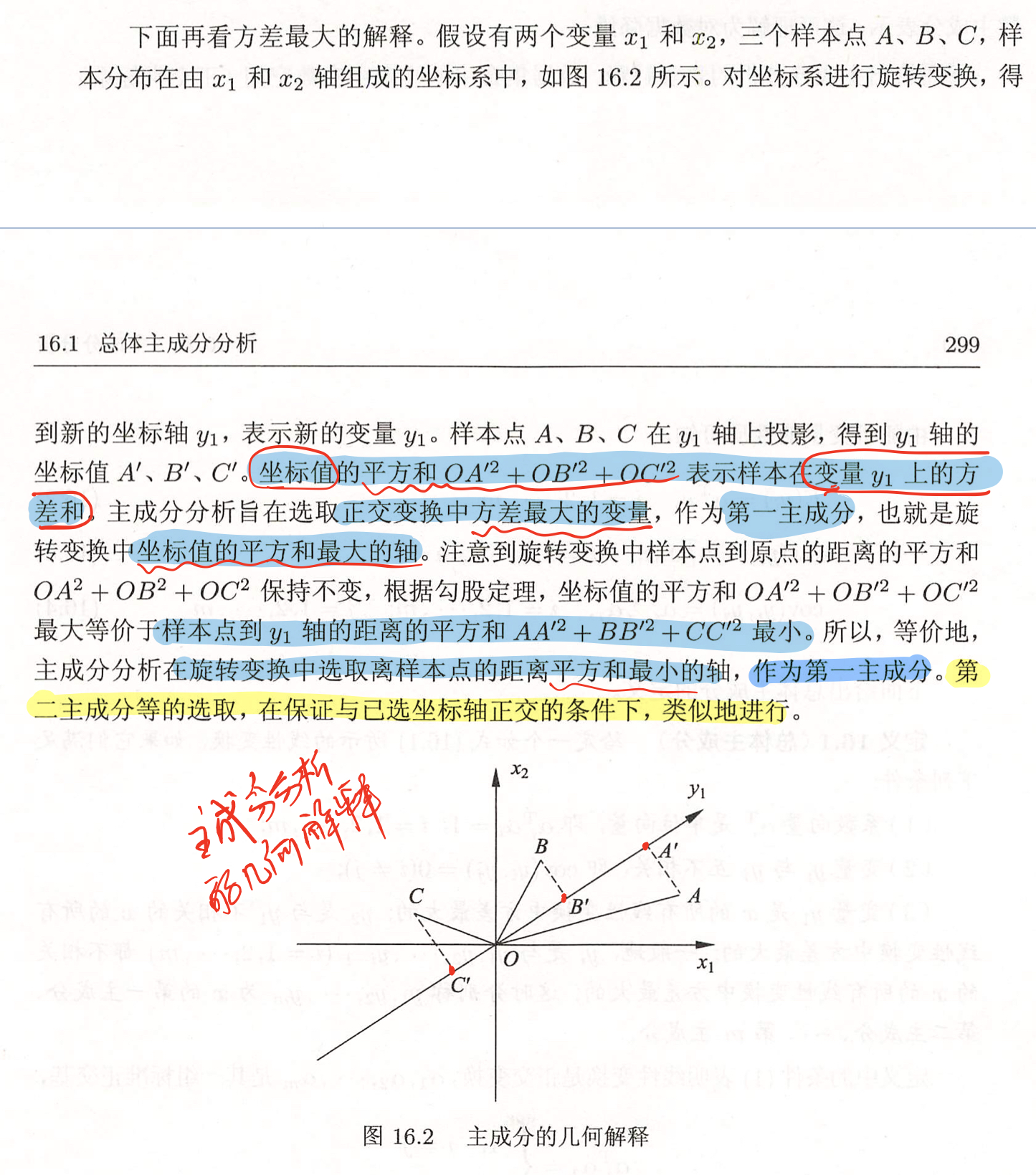总体主成分分析

定理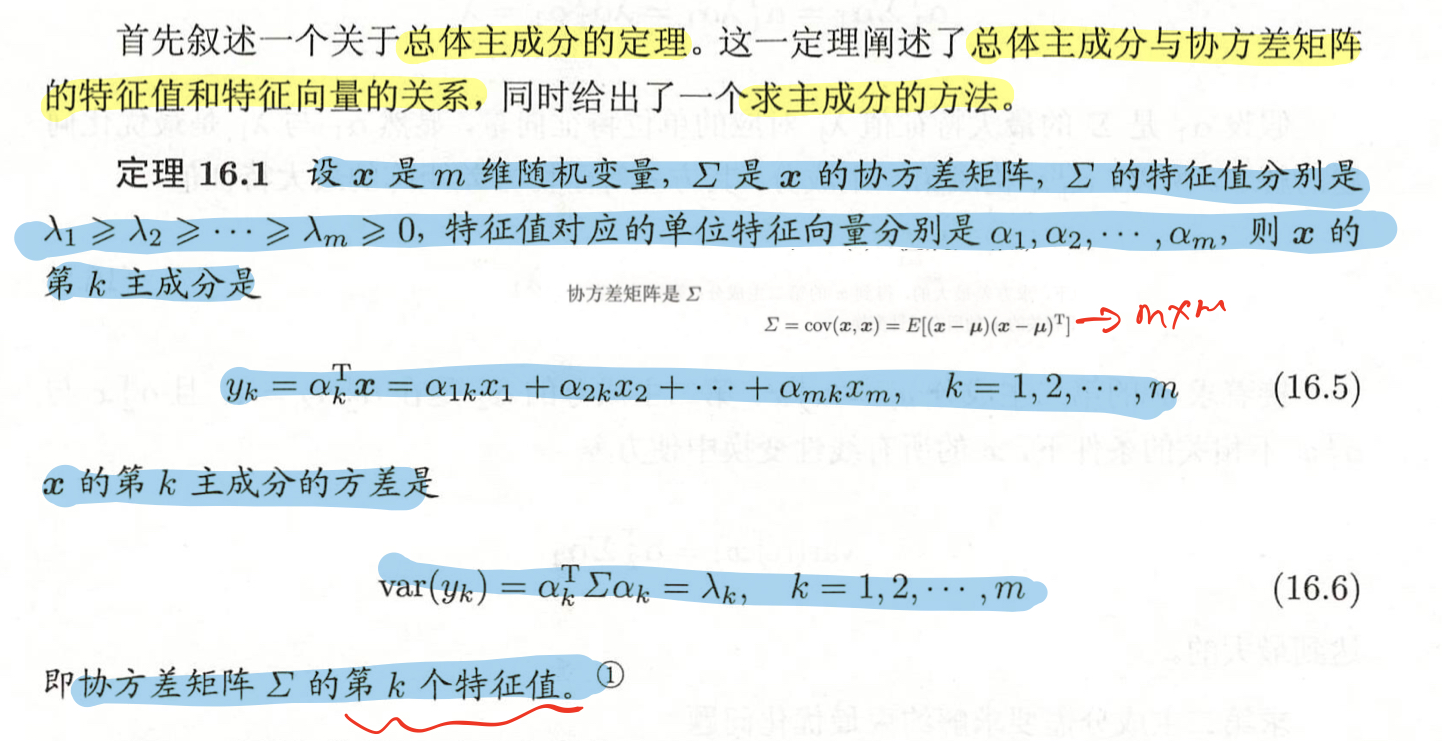规范化变量的总体主成分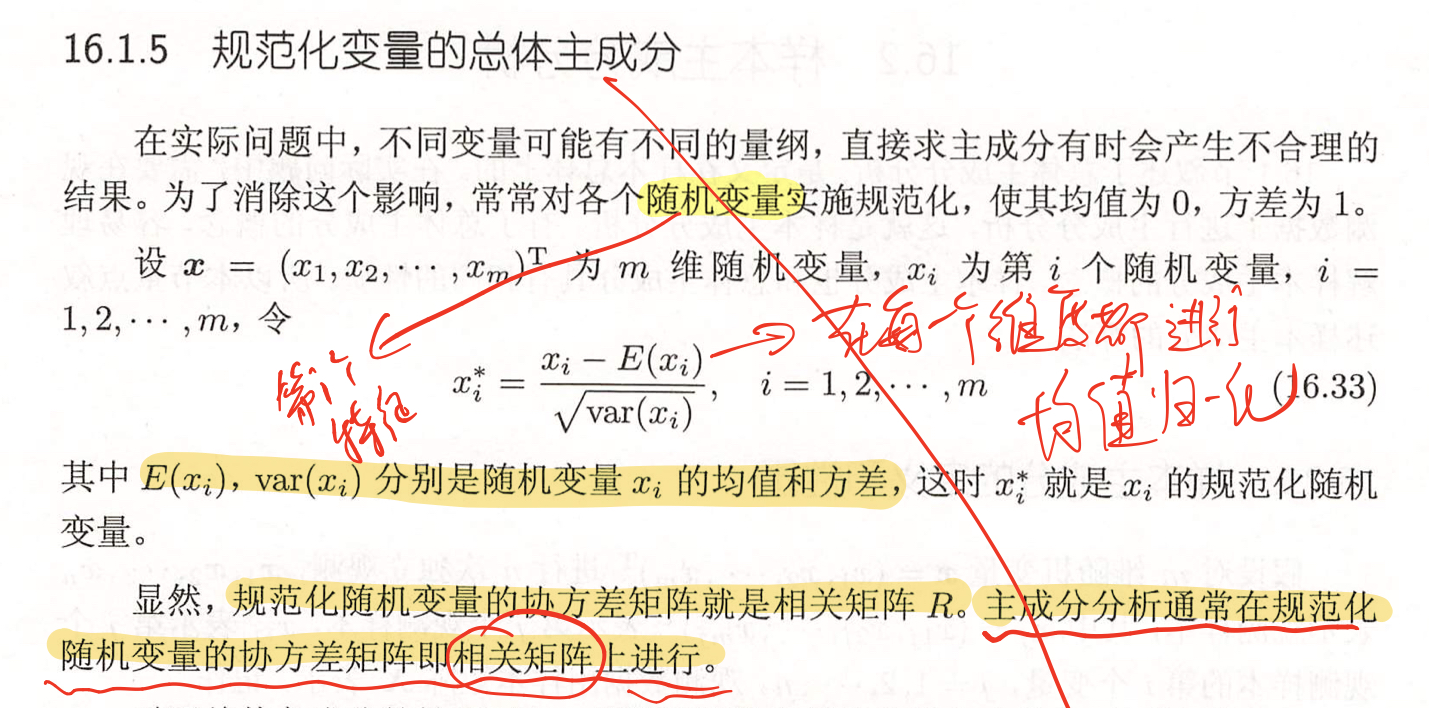样本主成分分析

定义和性质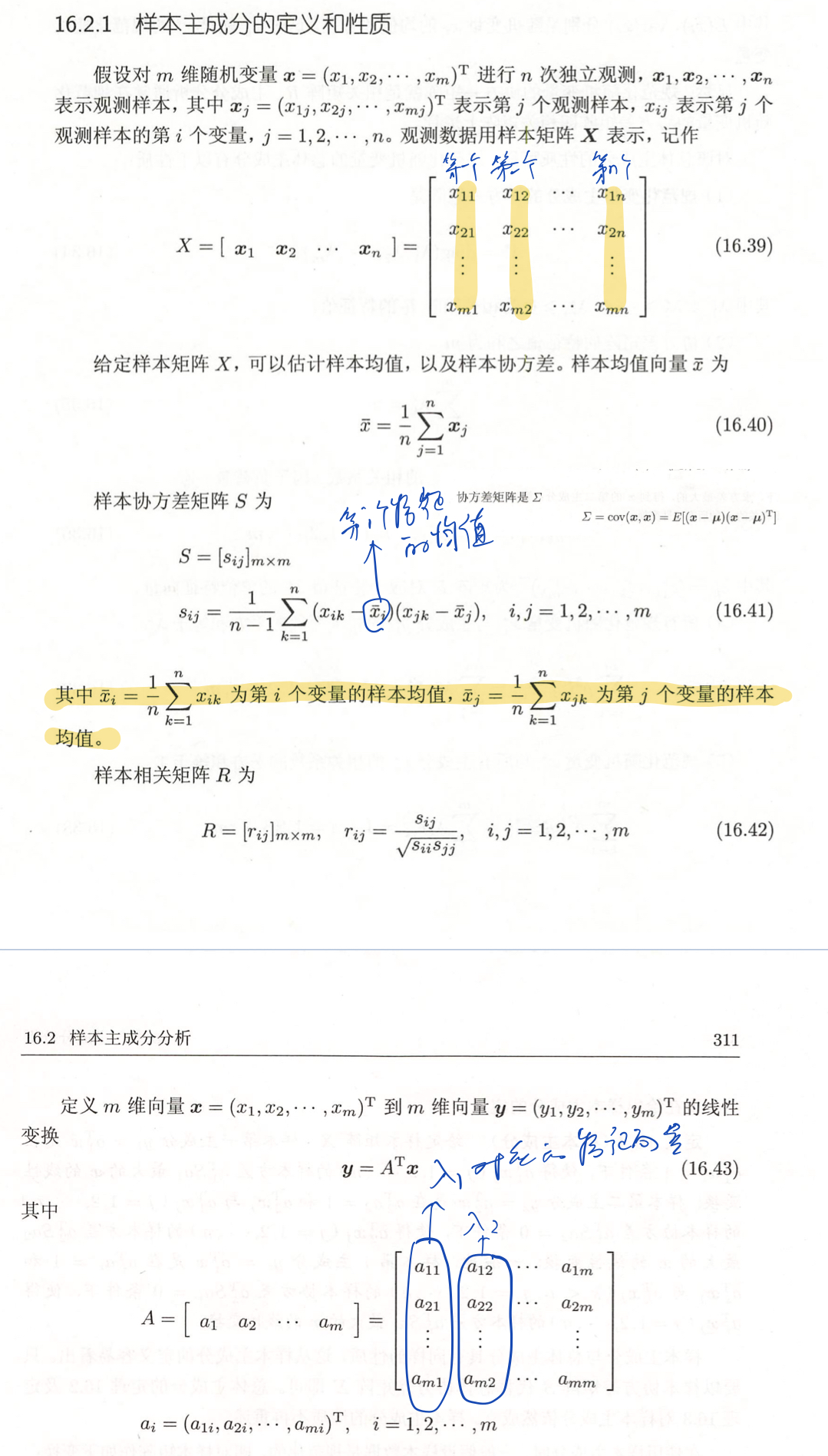变换后的协方差计算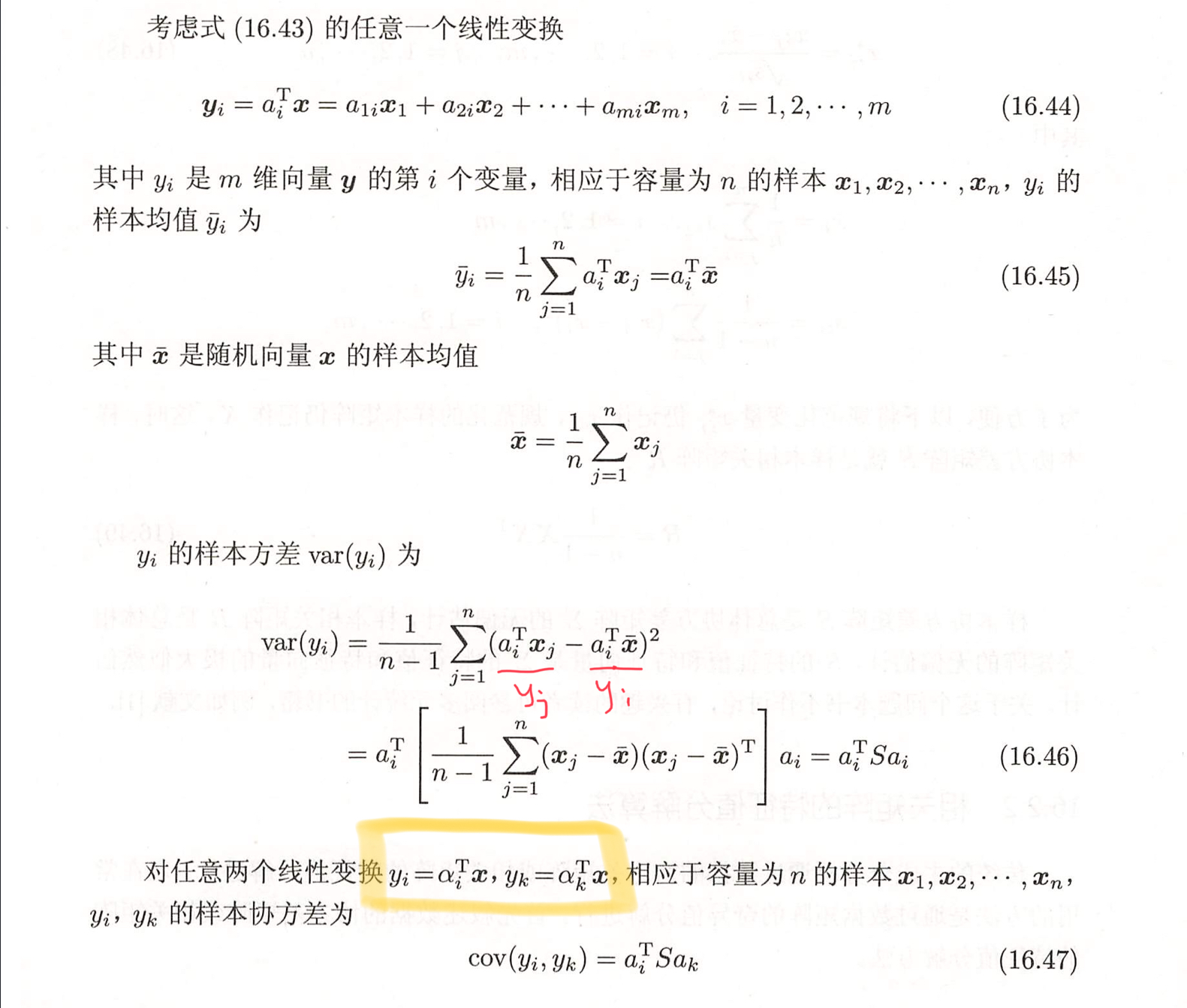相关矩阵的特征值分解算法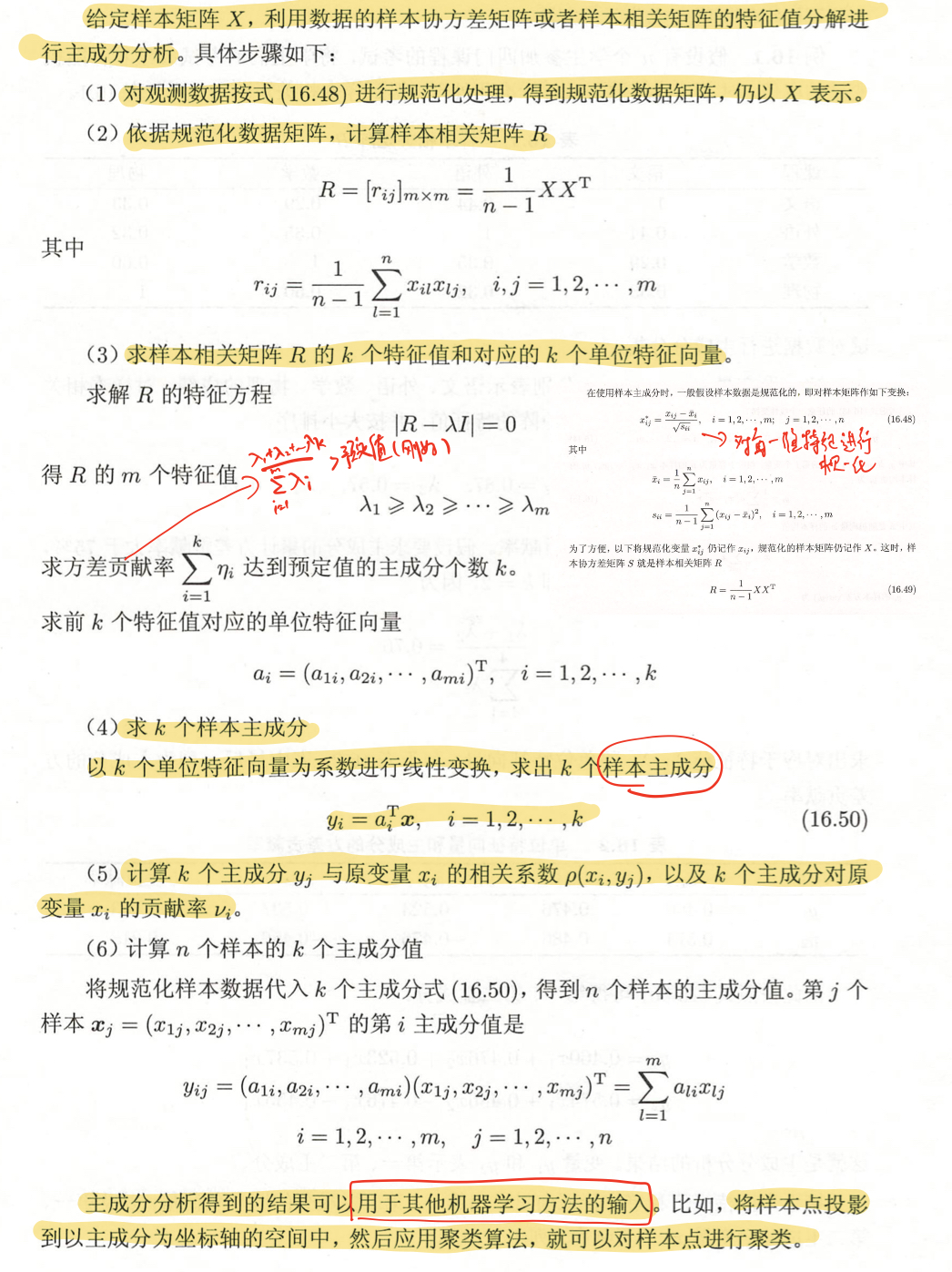数据矩阵的奇异值分解算法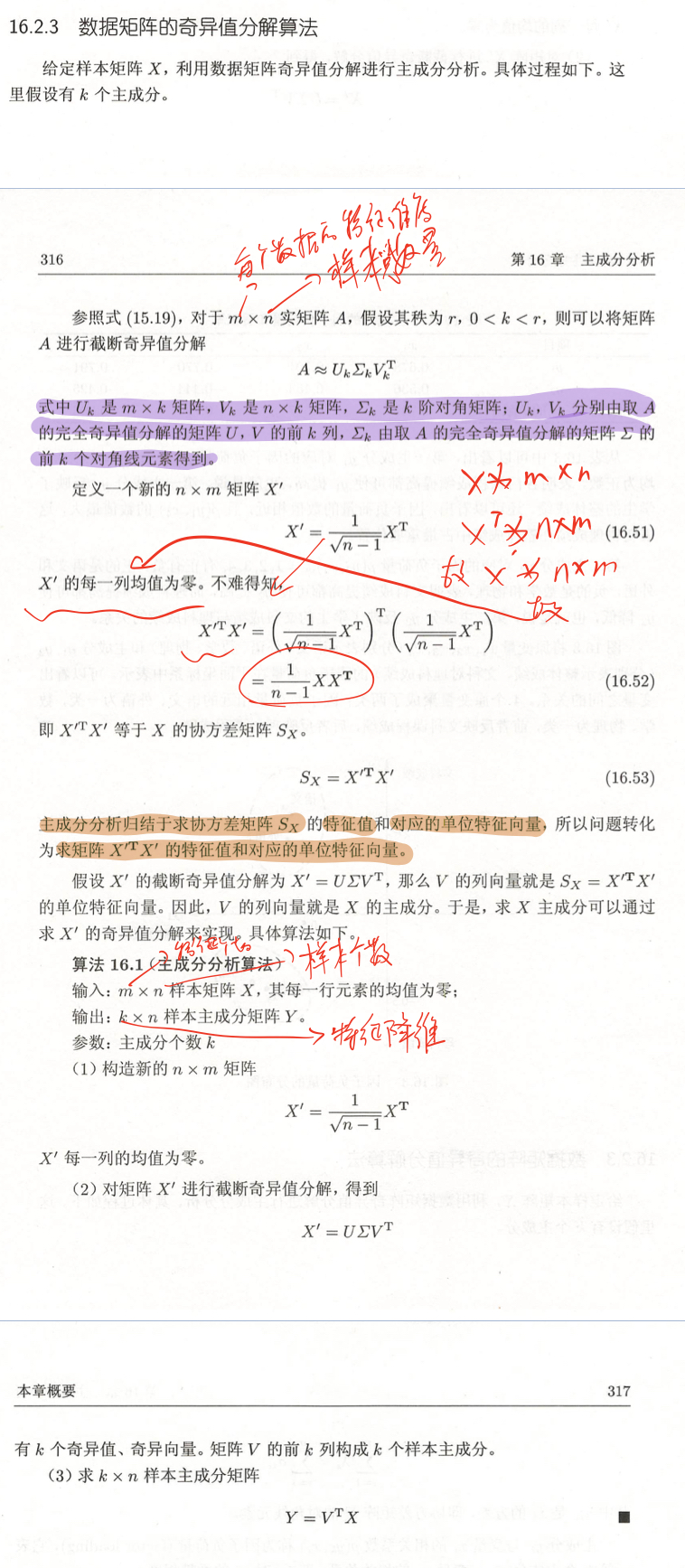总结

x的第i主成分的方差是协方差矩阵$\Sigma$的第i个特征值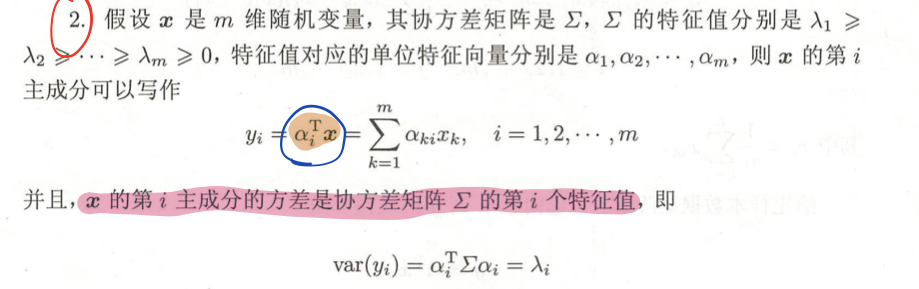主成分有以下性质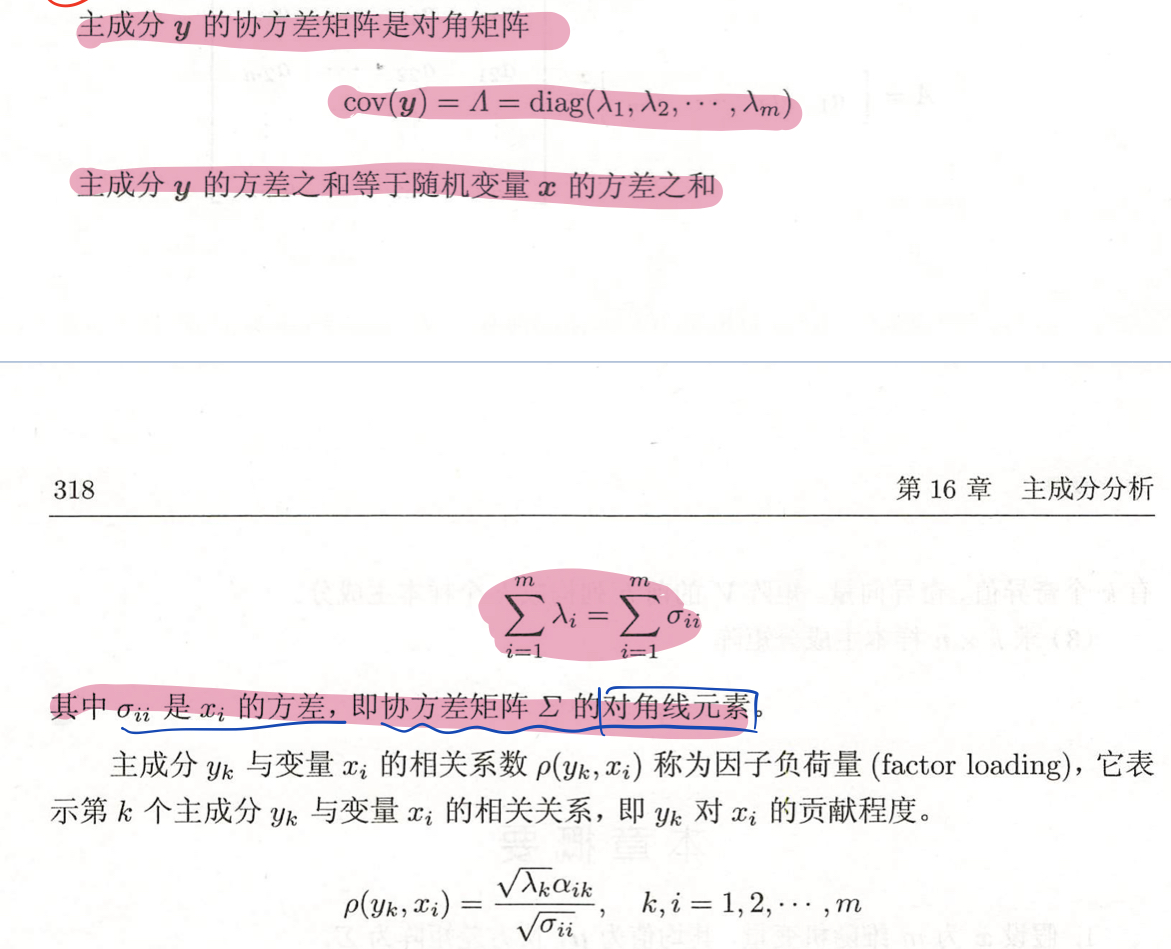样本主成分分析就是基于样本协方差矩阵的主成分分析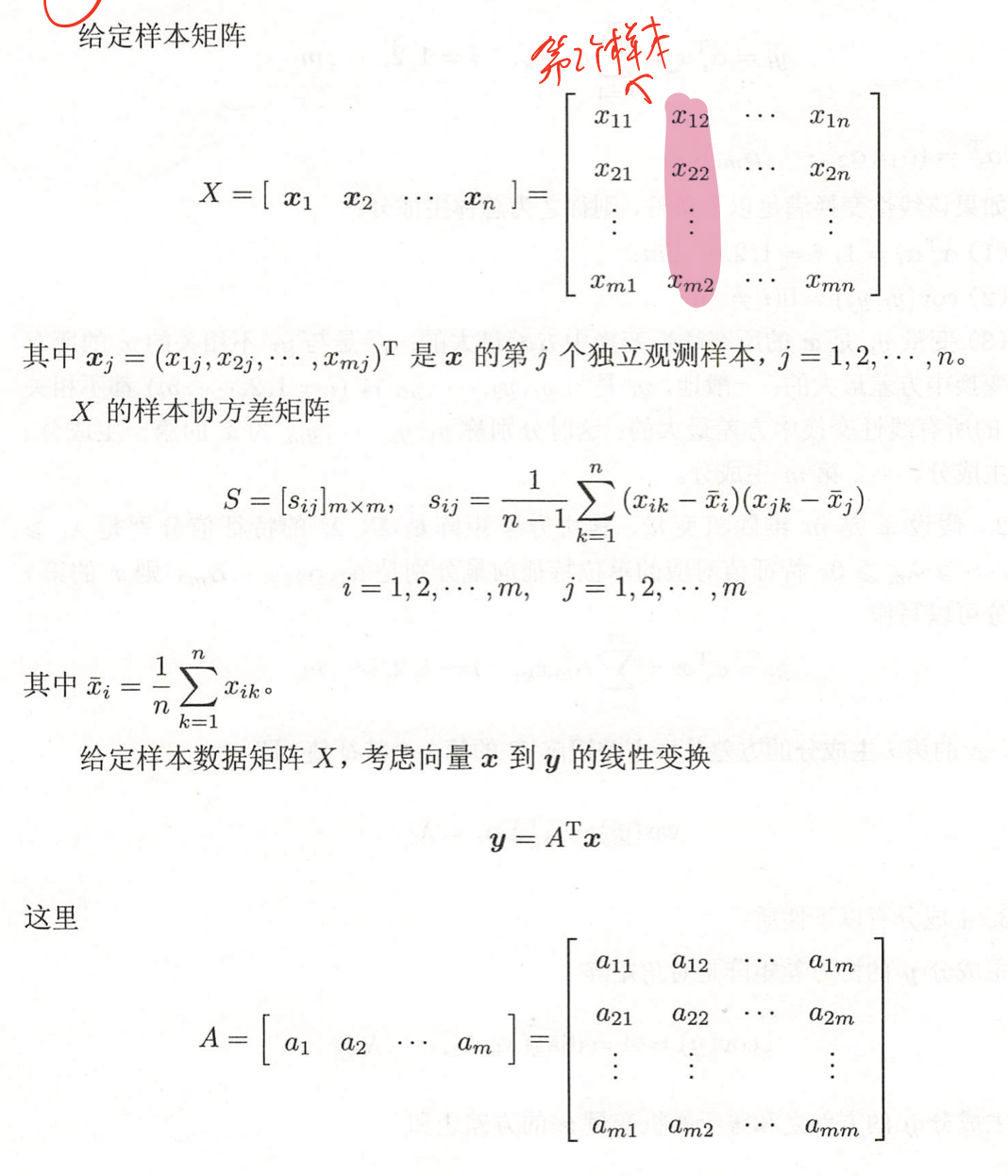两种主成分分析方法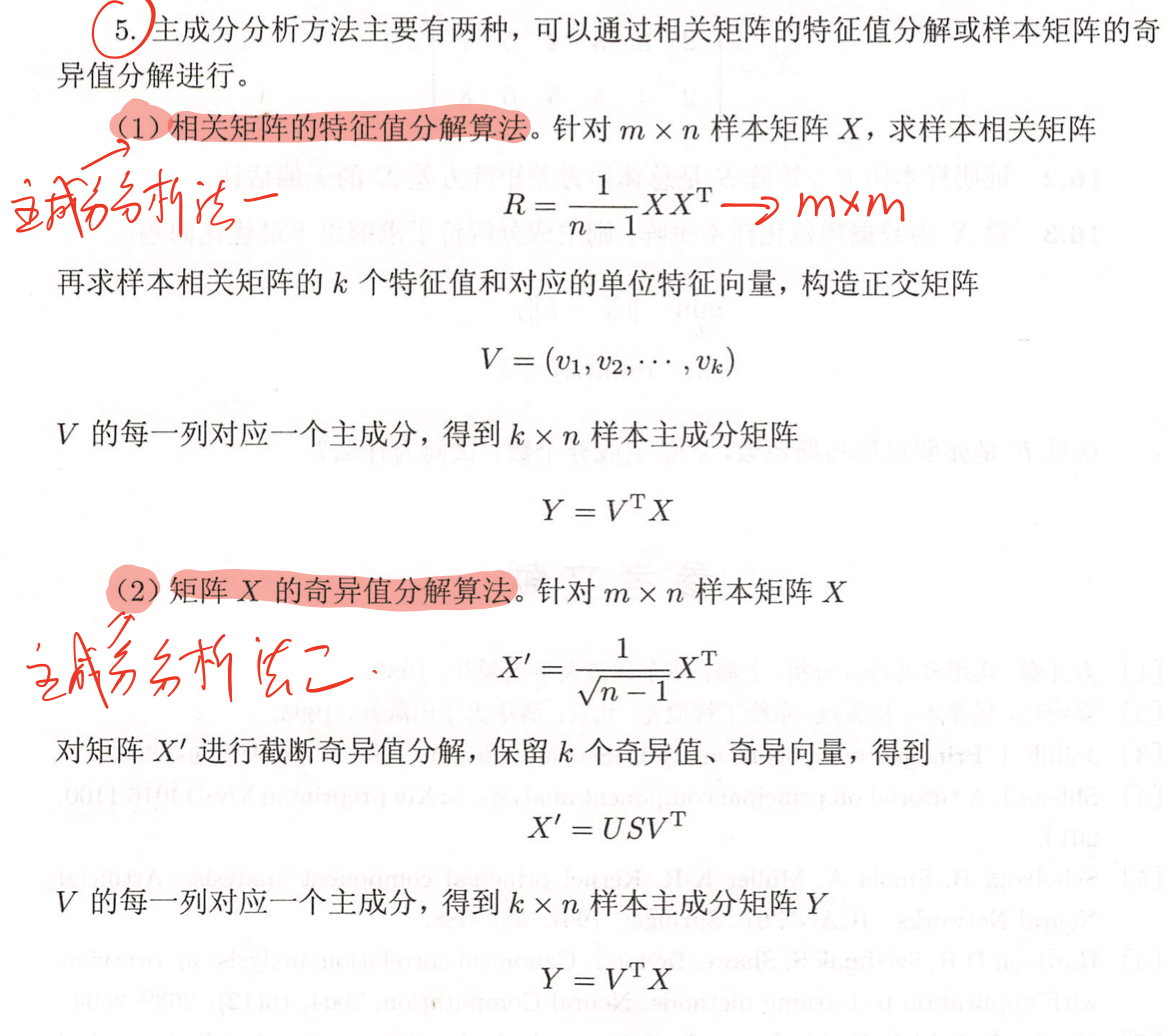展开全文• 目录1 总体主成分分析1.1 基本想法1.2 定义和导出1.3 主要性质1.4 主成分的个数1.5 规范化变量的总体主成分2 样本主成分分析2.1 样本主成分2.2 相关矩阵的特征值分解方法2.3 数据矩阵的奇异值分解算法总结 1 总体...pca降维
• 16 主成分分析16.1 总体主成分分析16.1.1 基本想法16.1.2 定义和导出16.1.3 主要性质 16.1 总体主成分分析 16.1.1 基本想法 数据变量之间可能存在相关性，以致增加了分析难度。于是，考虑由少数不相关变量来...机器学习
• §6.4 系统聚类法的性质及类的确定 §6.5 动态聚类法 §6.6 有序样品聚类法(最优分割法) §6.7 变量聚类方法 习题六 第七章 主成分分析 §7.1 总体主成分 §7.2 样本的主成分 §7.3 主成分分析的应用 习题七 第八...
• 0 目录1. 假设检验2. 卡方检验3. 方差检验4. 相关系数5. 线性回归6. 主成分分析PCA7....我们换一个说法来解释假设检验就是做出一个假设，然后根据数据或者已知分布性质来推断这个假设成立概率有多大，具体...
• 　3.3 极大似然估计及估计量的性质 　3.4 〖WTHX〗〖Akx-〗和／n-1)S的抽样分布 *§3.5 二次型分布 小结 附录3-1 SAS的应用 附录3-2 §3.2中若干性质的数学证明 习题 第四章 多元正态总体的统计推断 　4.1 一元情形...